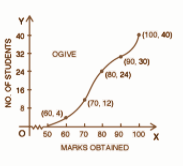# West Bengal Madhyamik Board Question Paper for Class 10th Maths 2018 In PDF

West Bengal Madhyamik (Class 10) Maths 2018 question paper with solutions are provided on this page by BYJU’S in downloadable pdf format and also in the text, so that the students can make use of the given model papers and test where they stand in terms of their preparation level. Students can practise the West Bengal Madhyamik (Class 10) Maths 2018 question paper to ace the exams.

Maths Question Paper 2018 Class 10 is added here, which introduces the students to the important concepts that have to be covered for their exams. Students are able to access all the West Bengal board previous year maths question papers. Students will get to know their weak areas which they can improve and at the same time have a quick revision of the entire syllabus.

### WBBSE Class 10th Maths Question Paper With Solutions 2018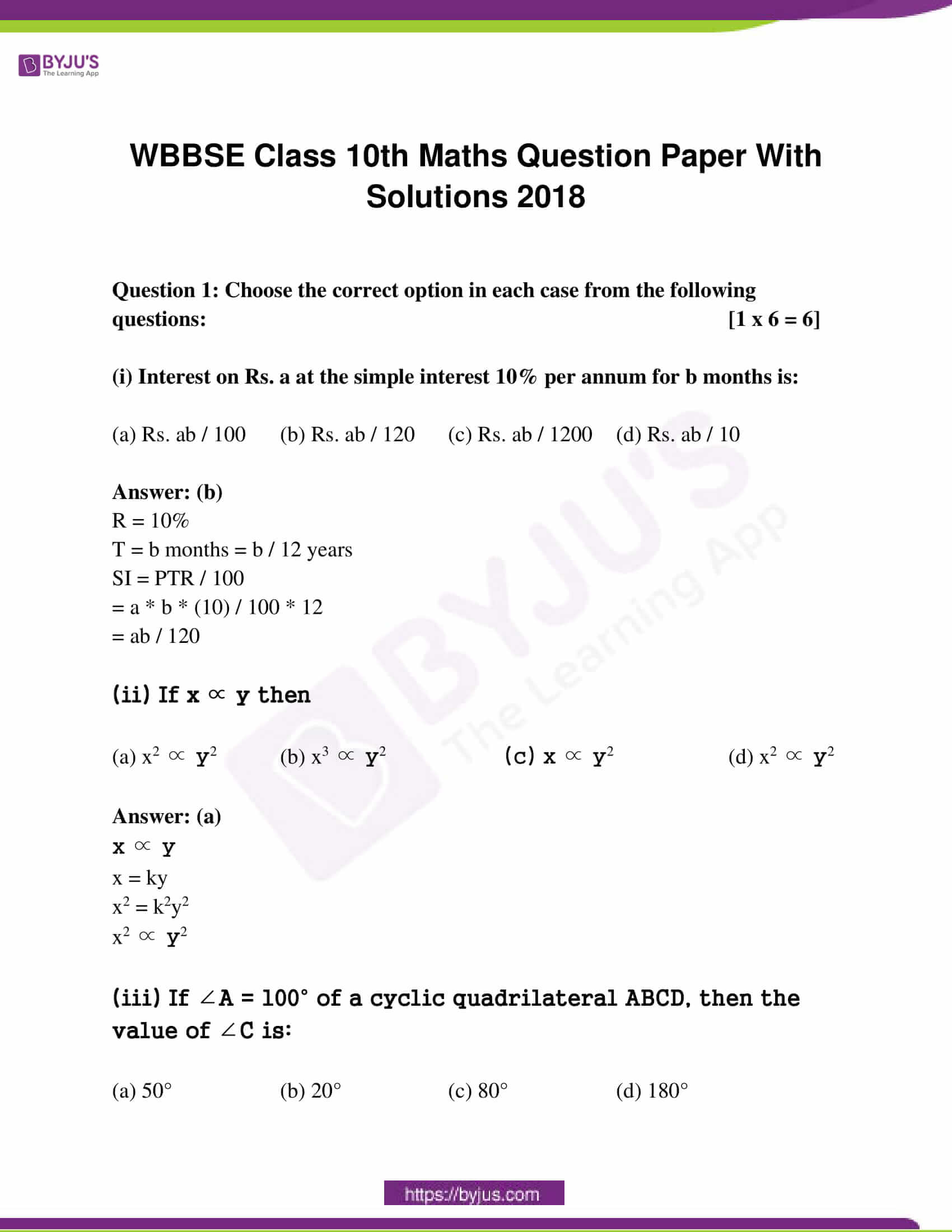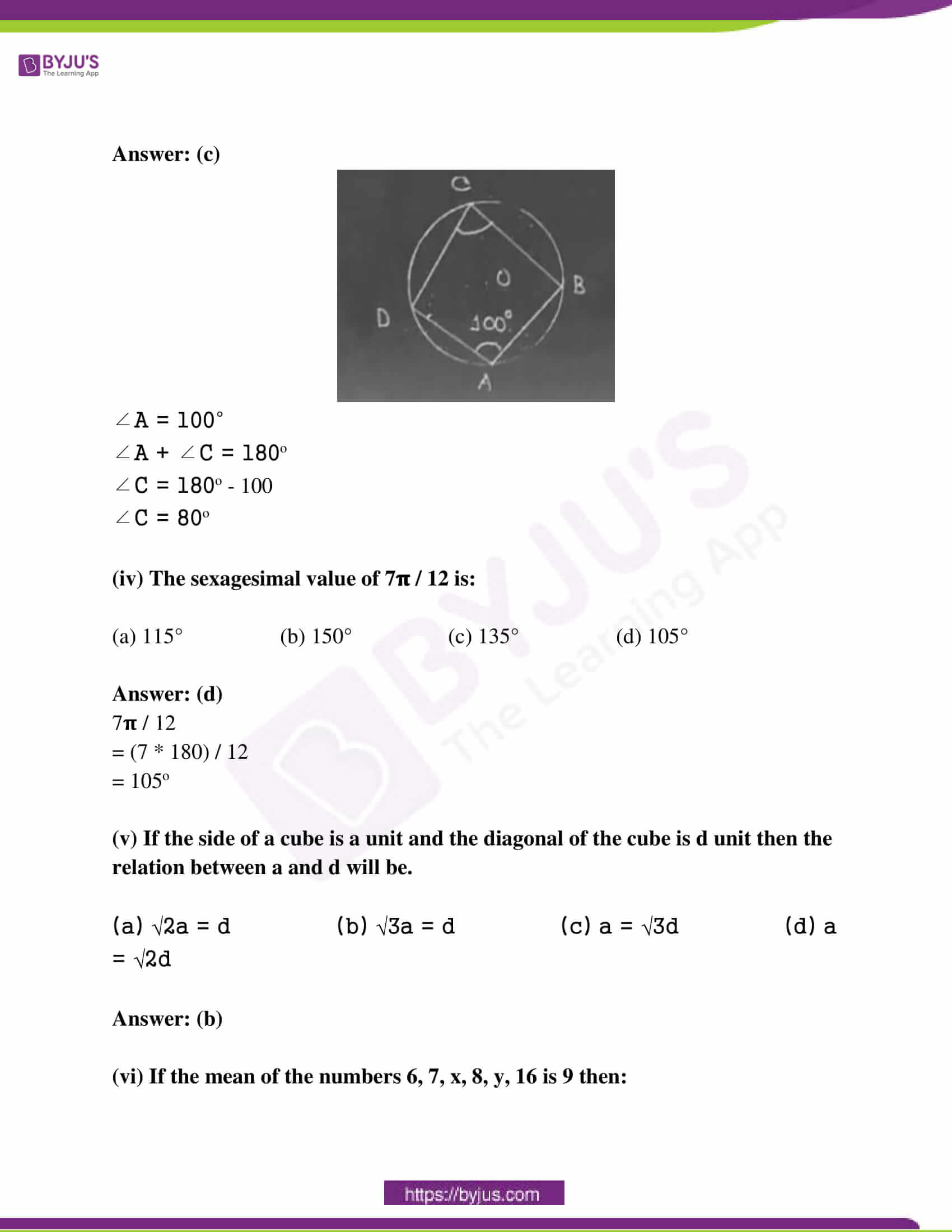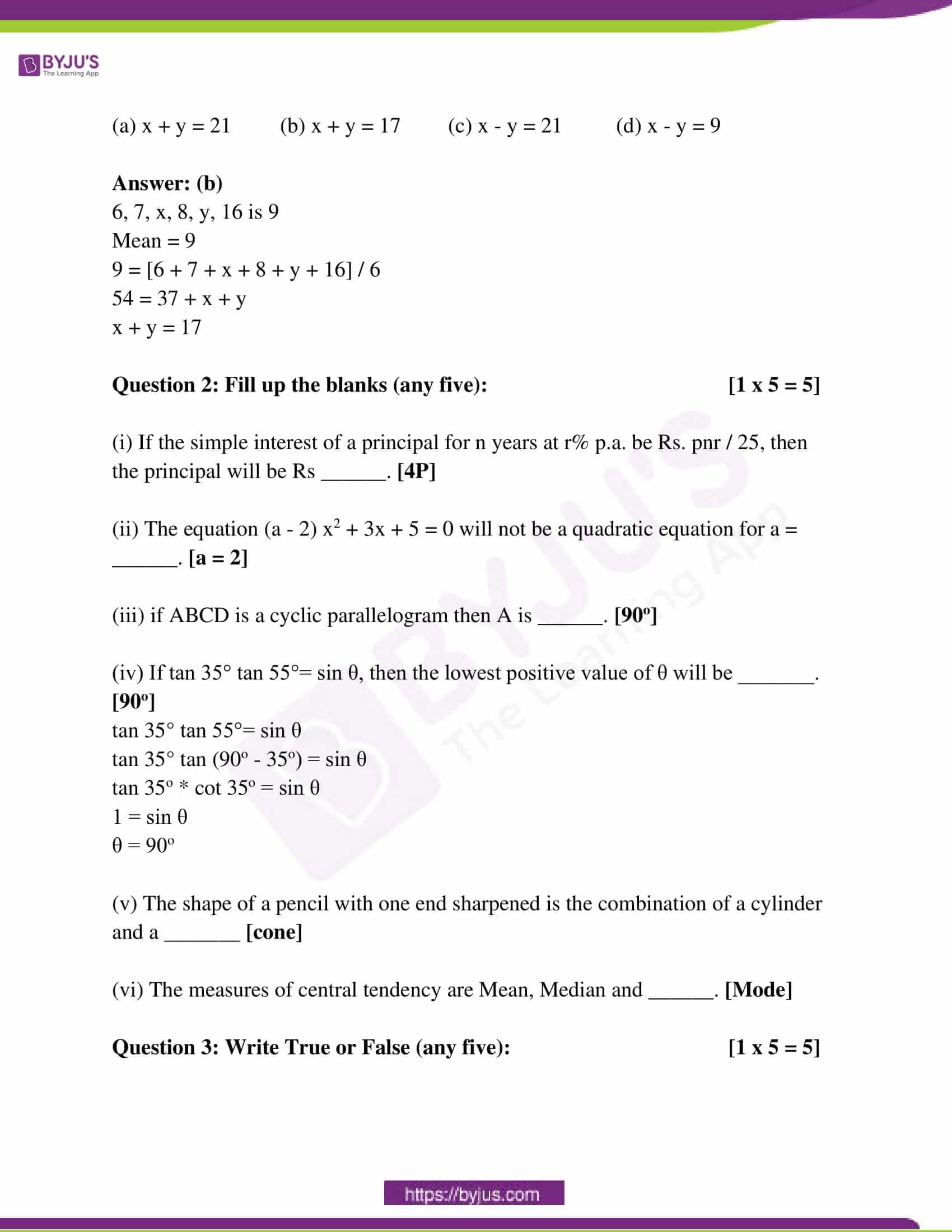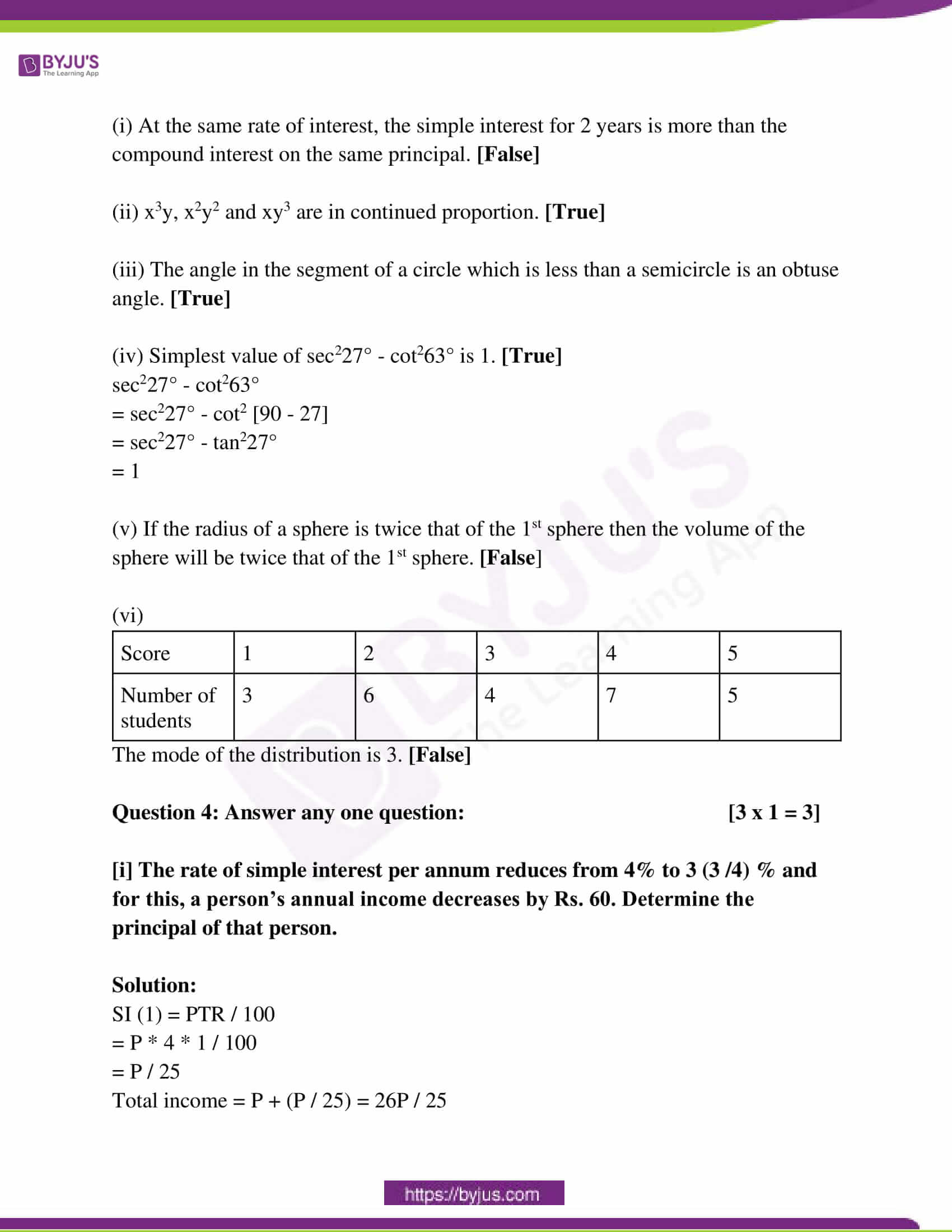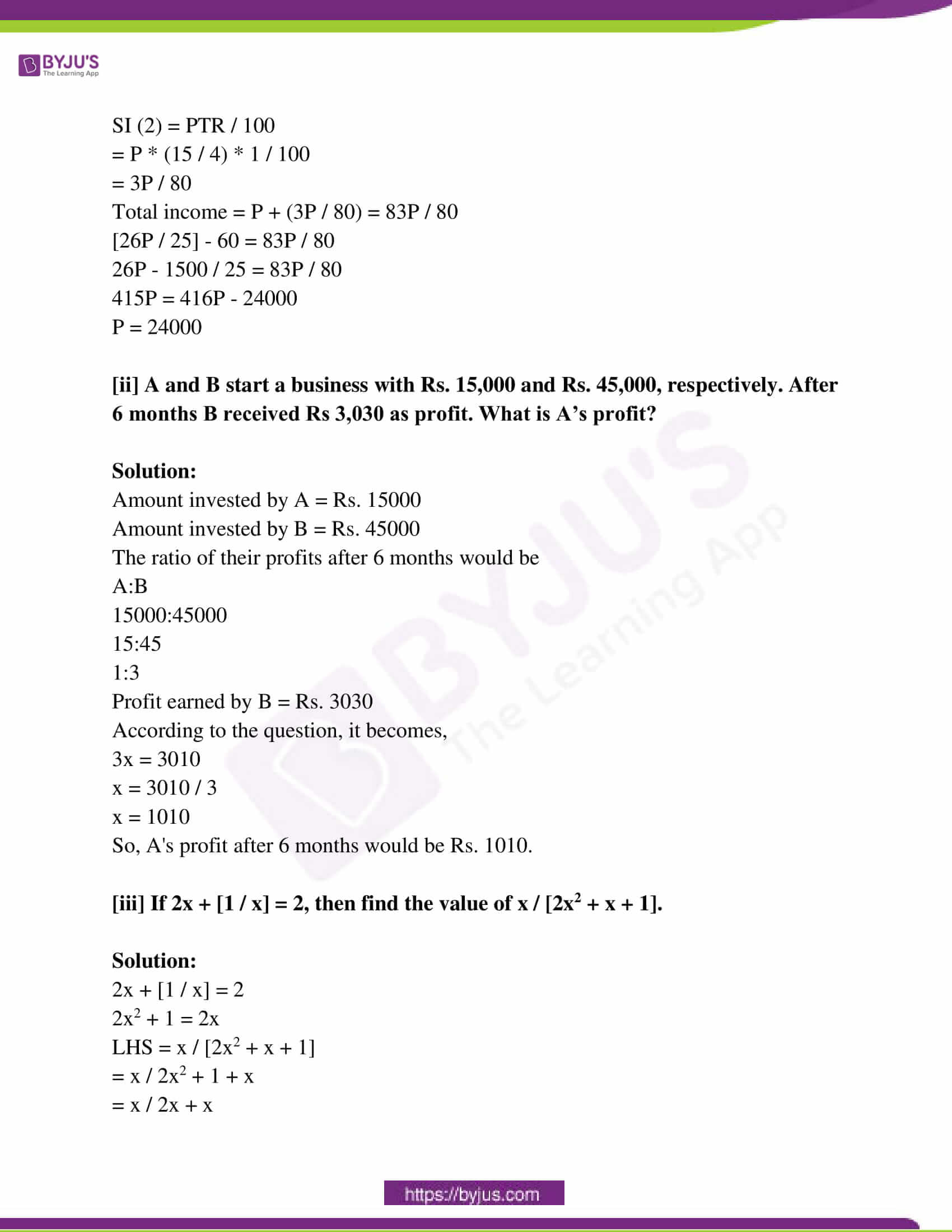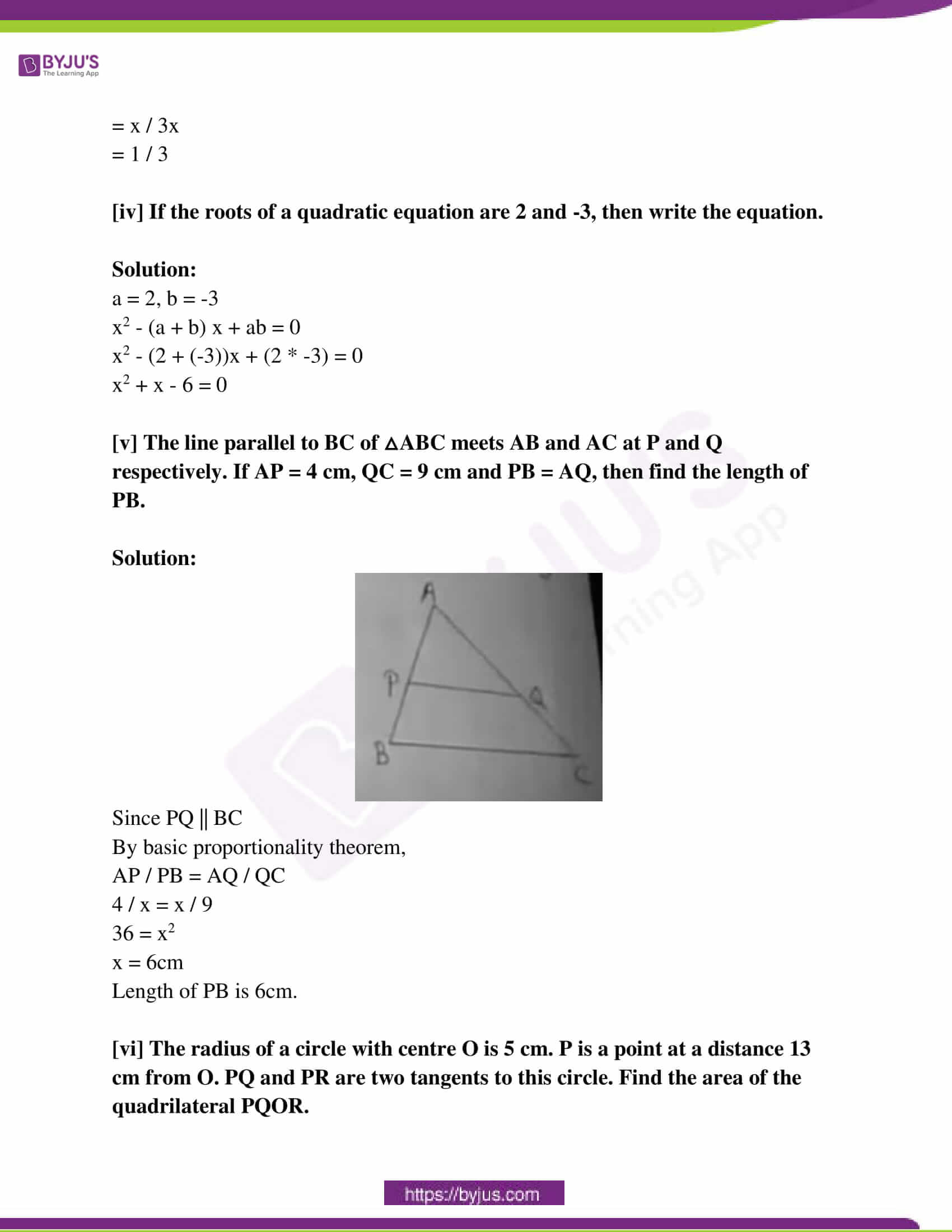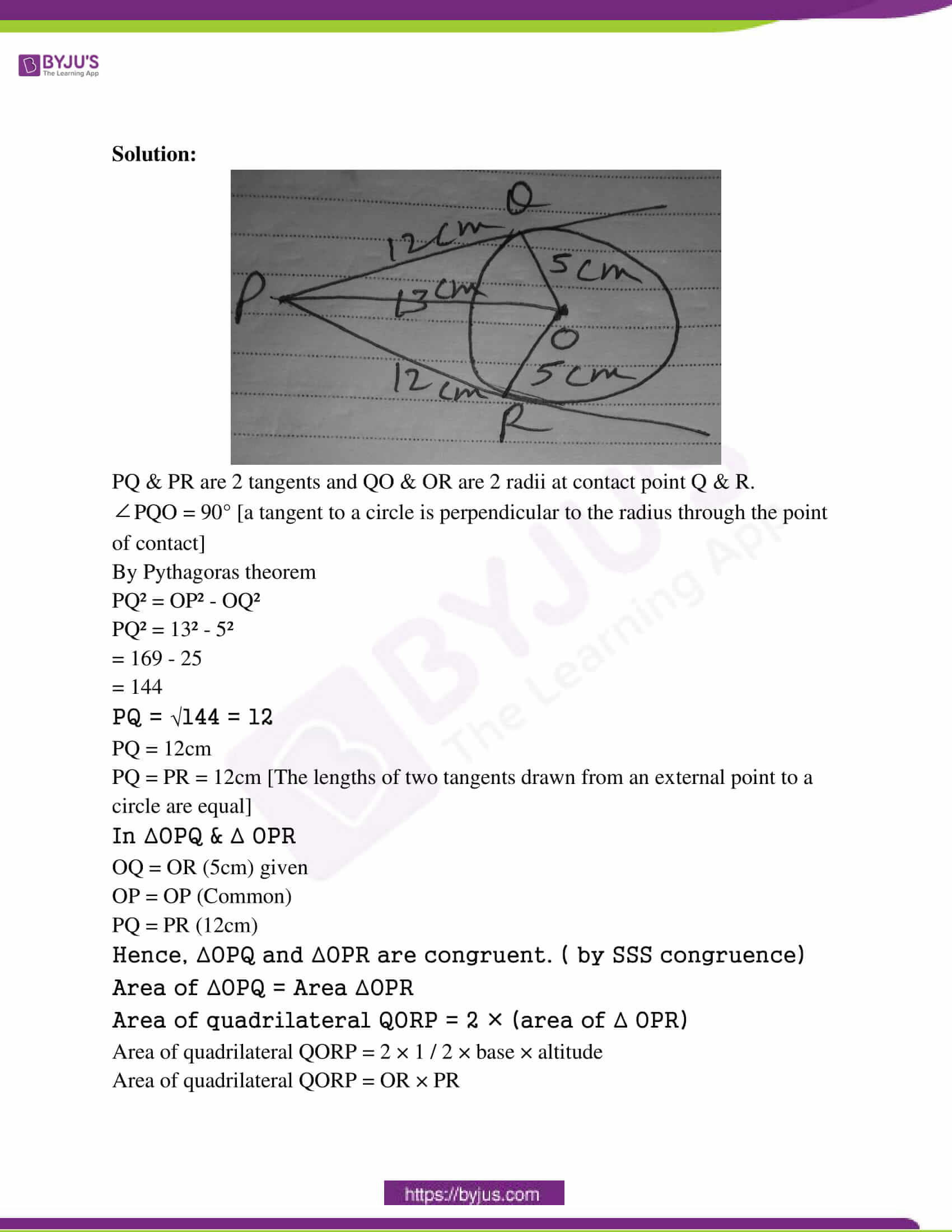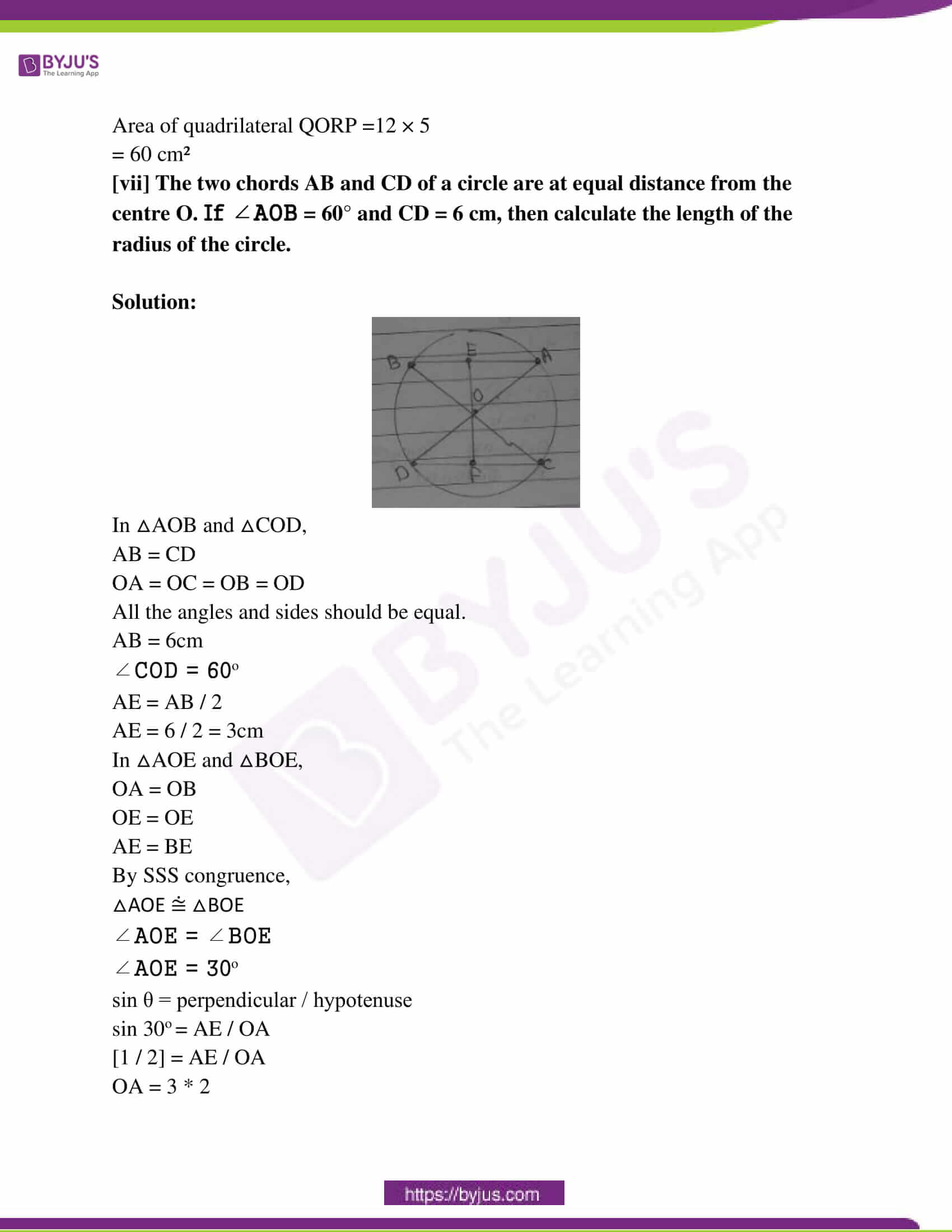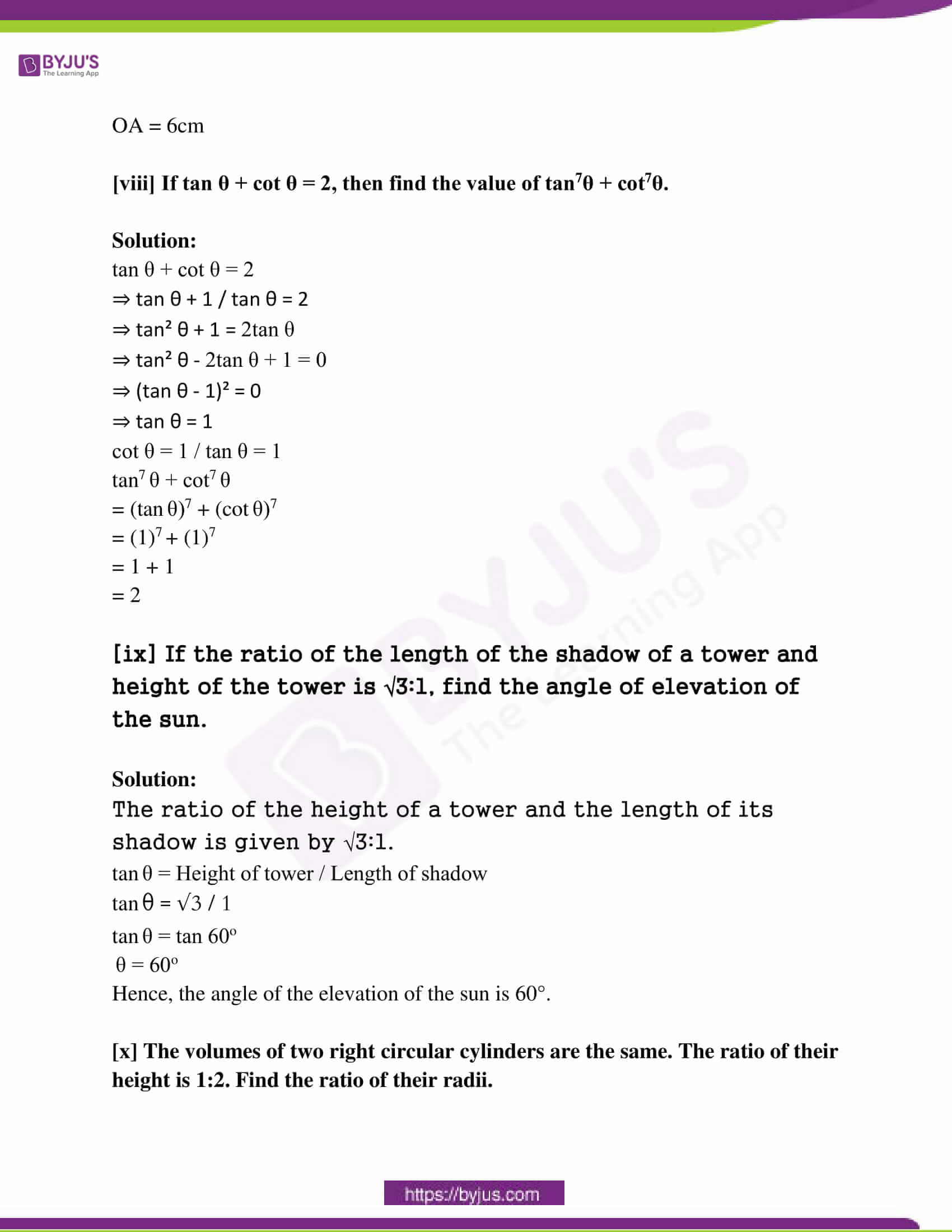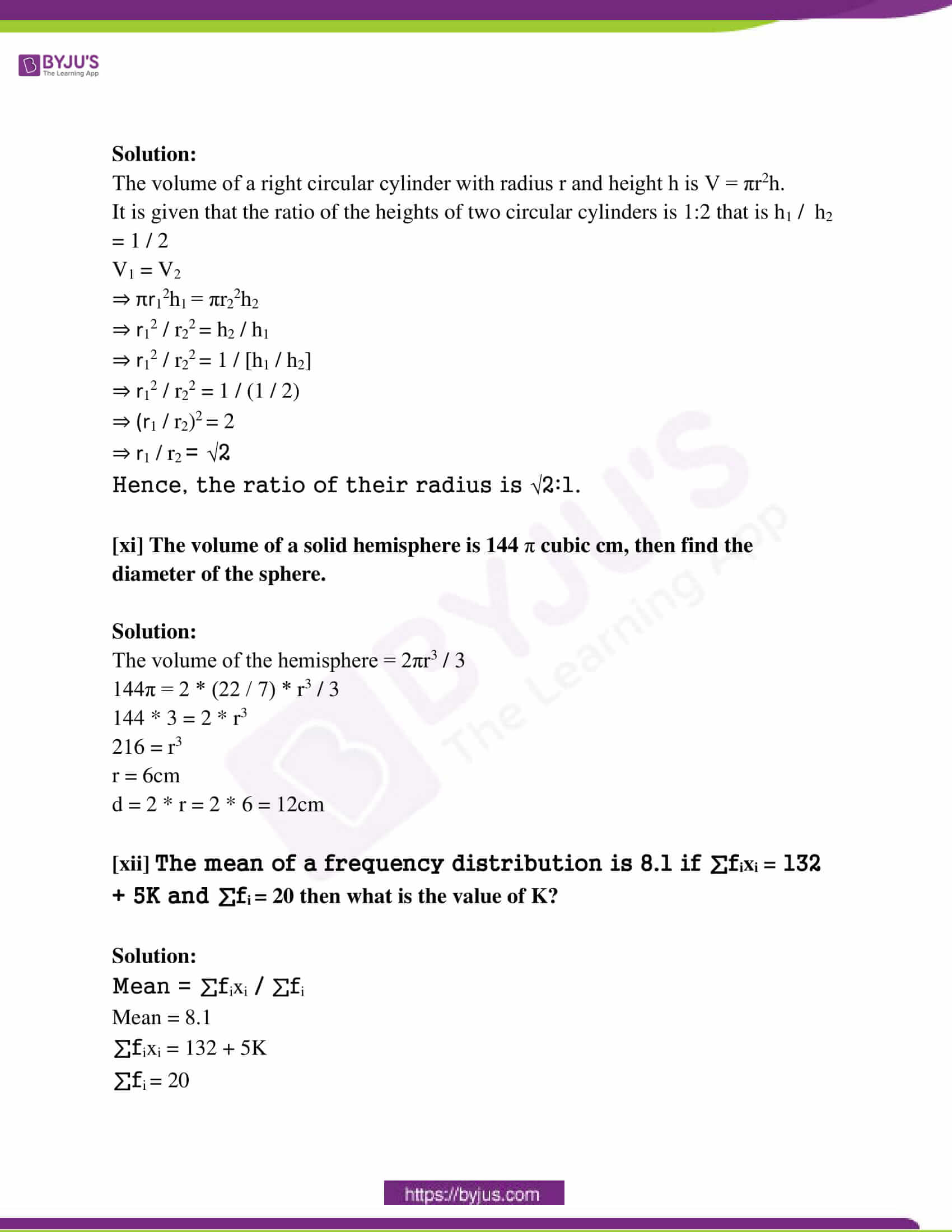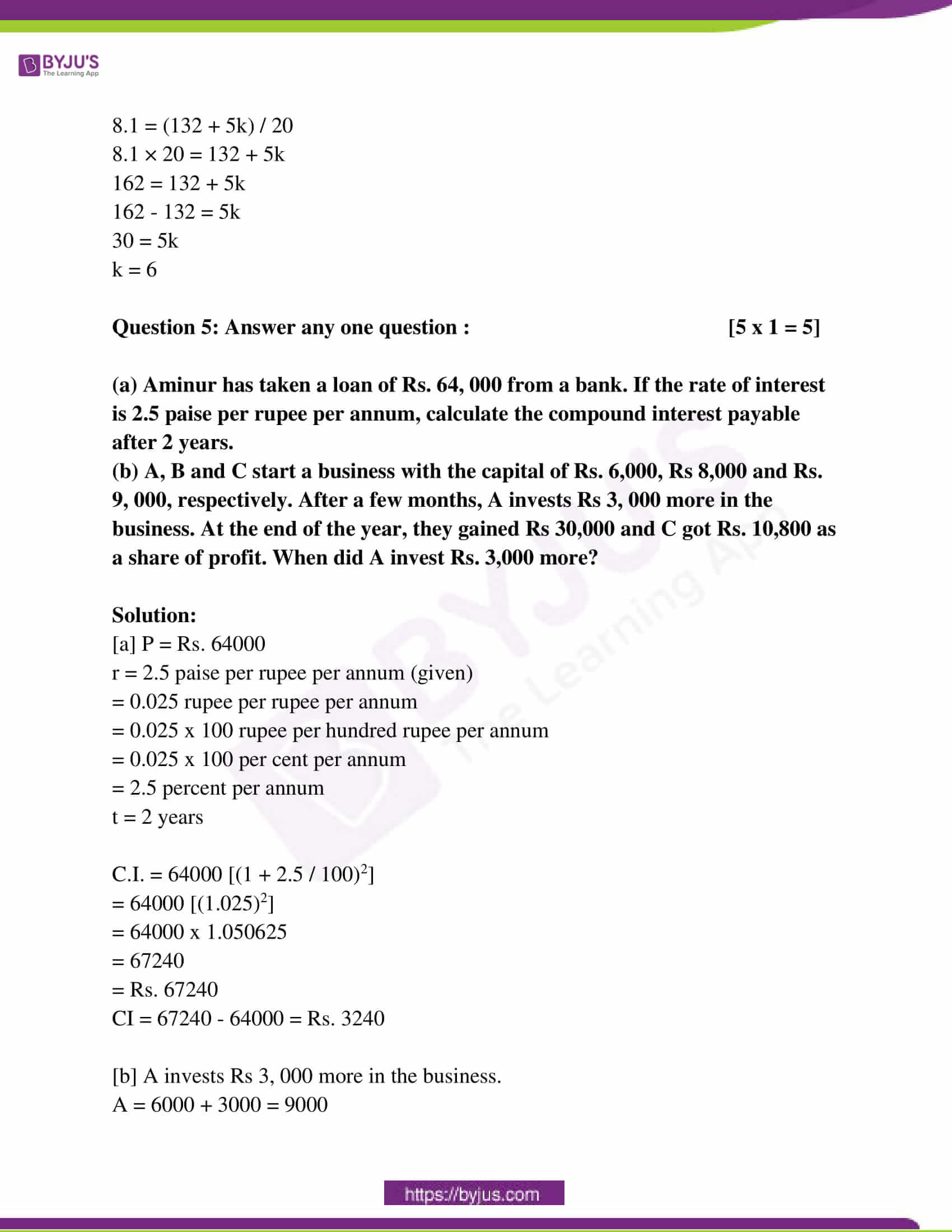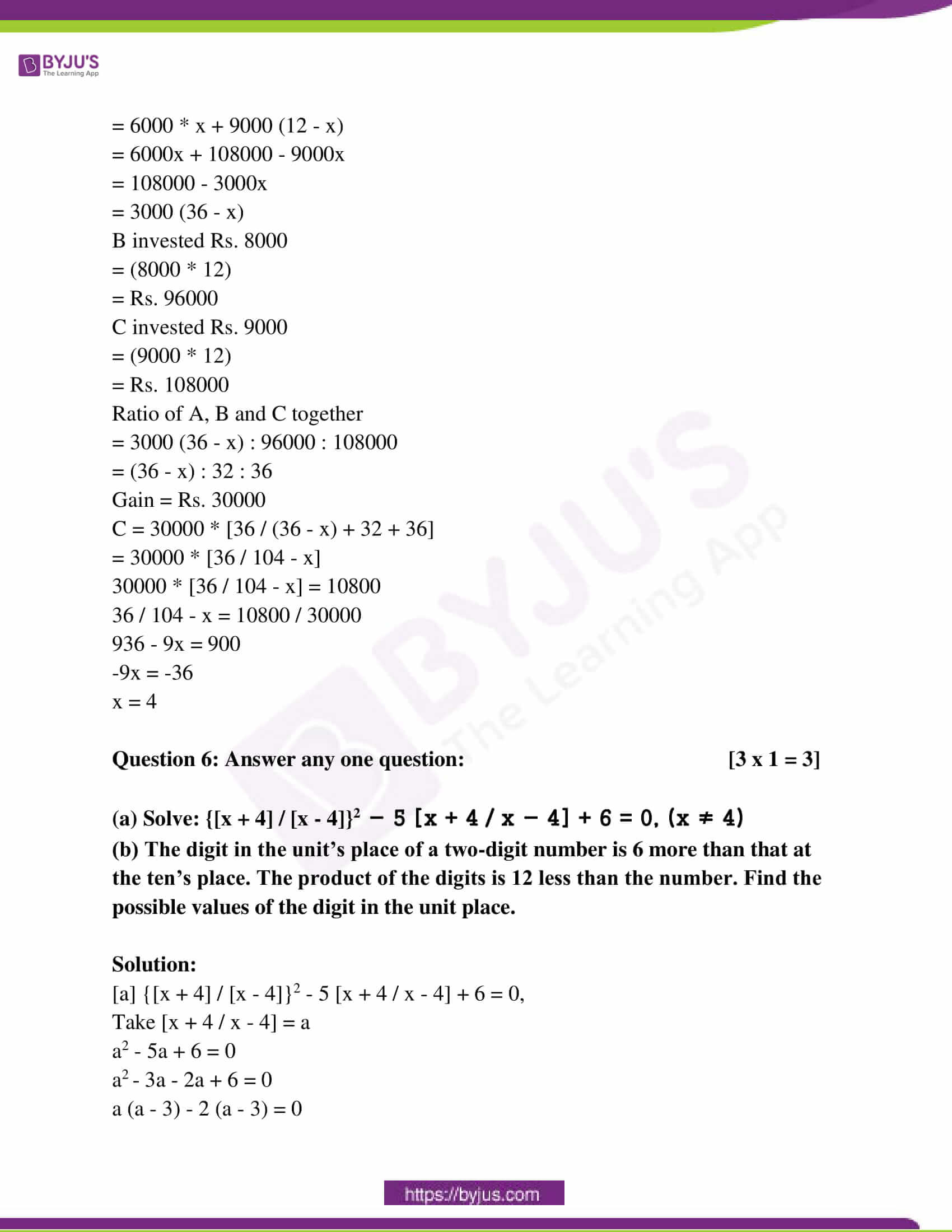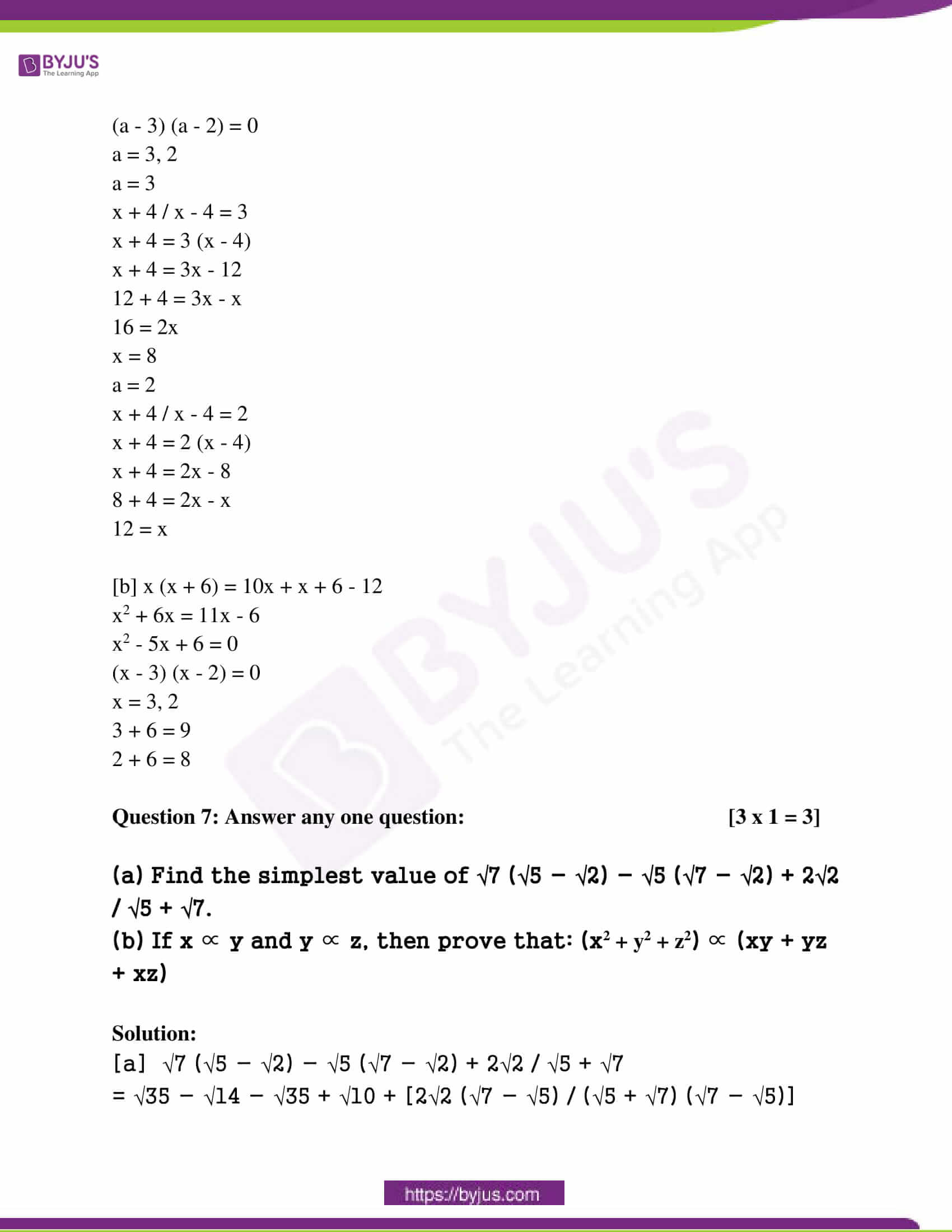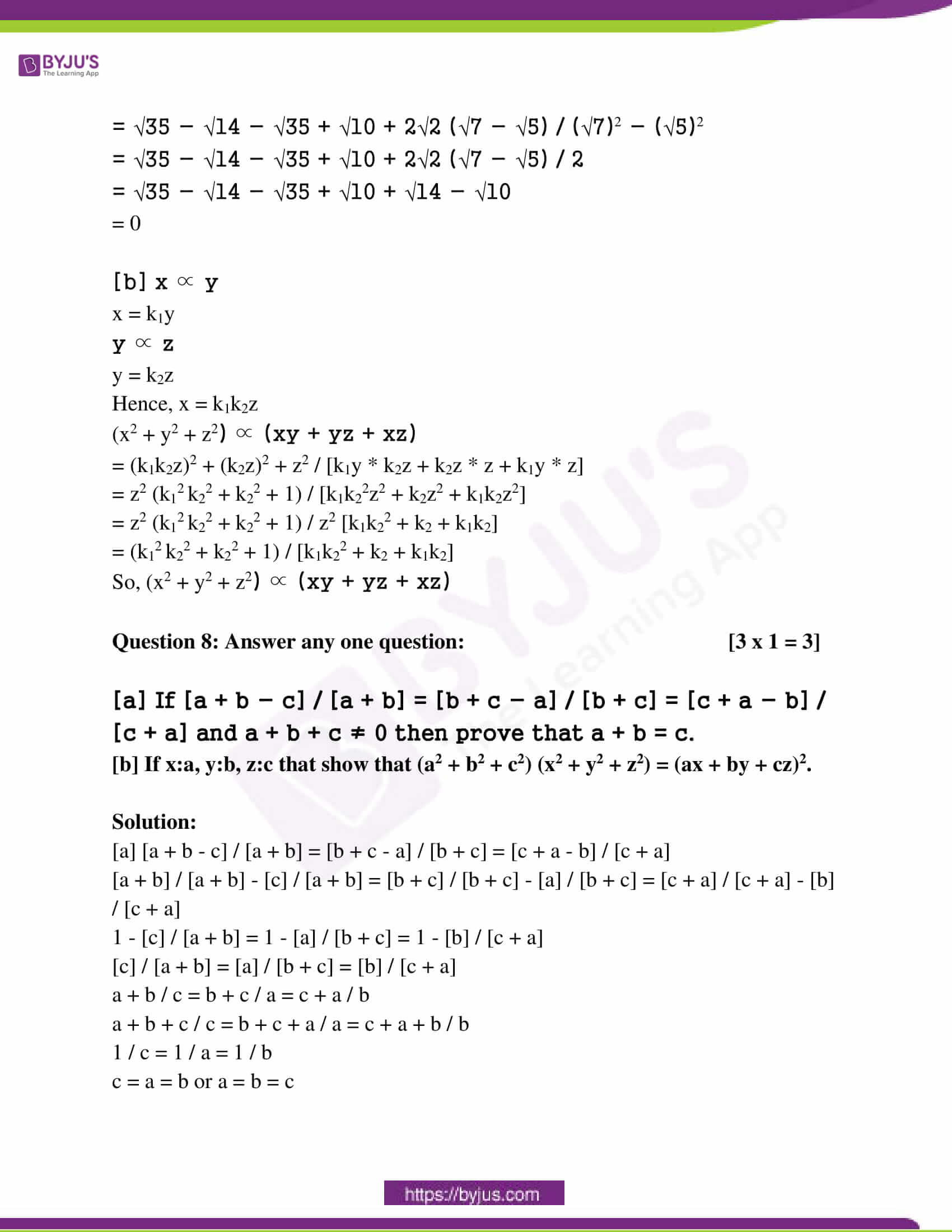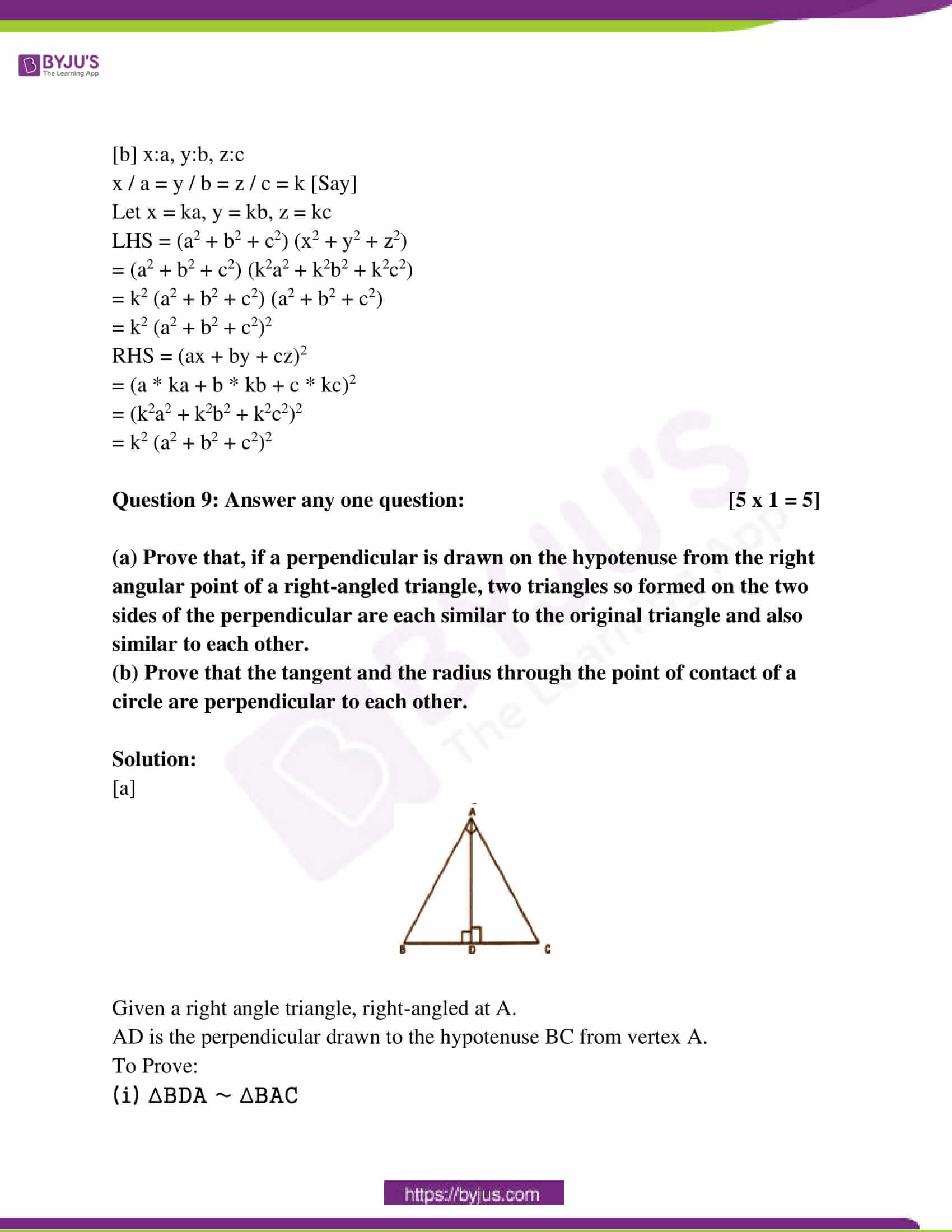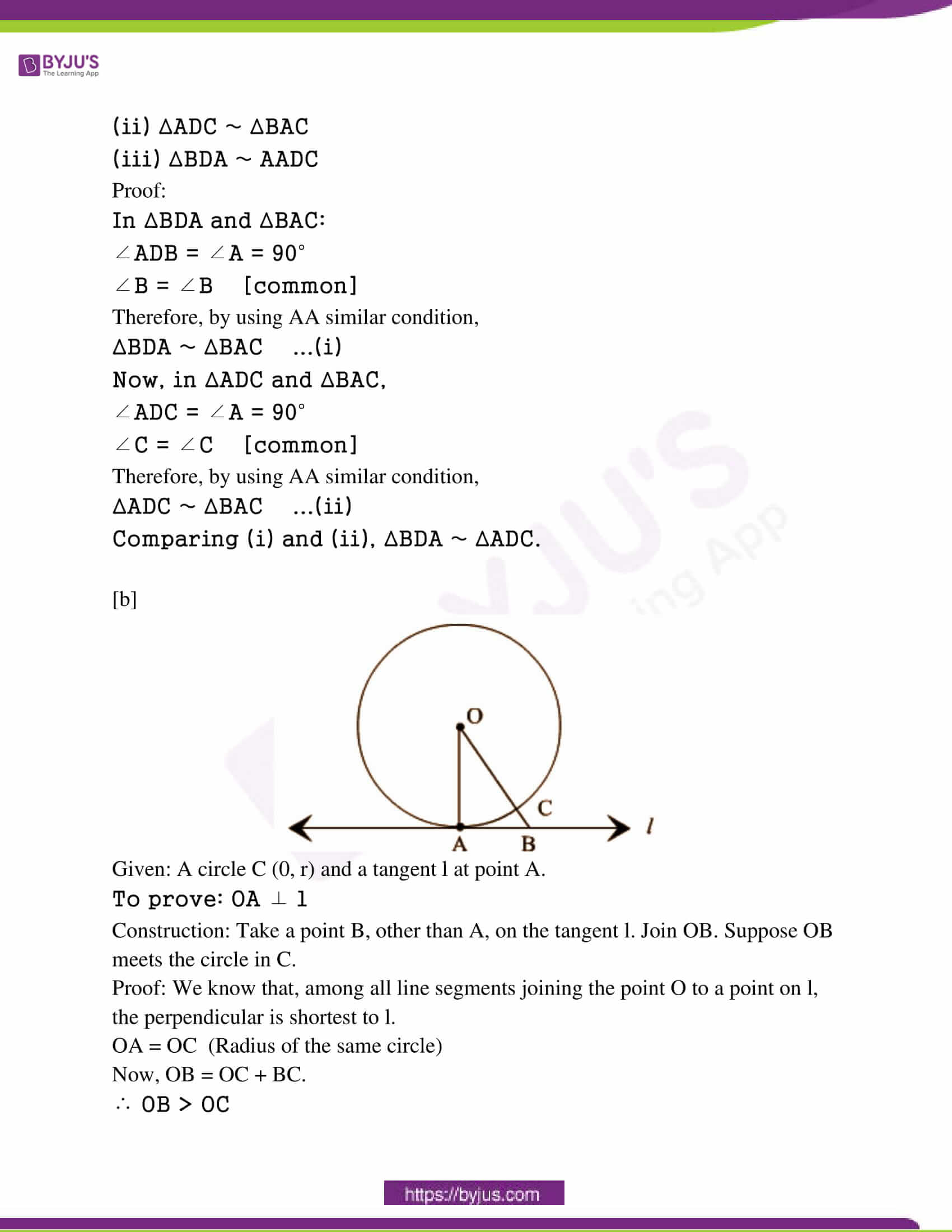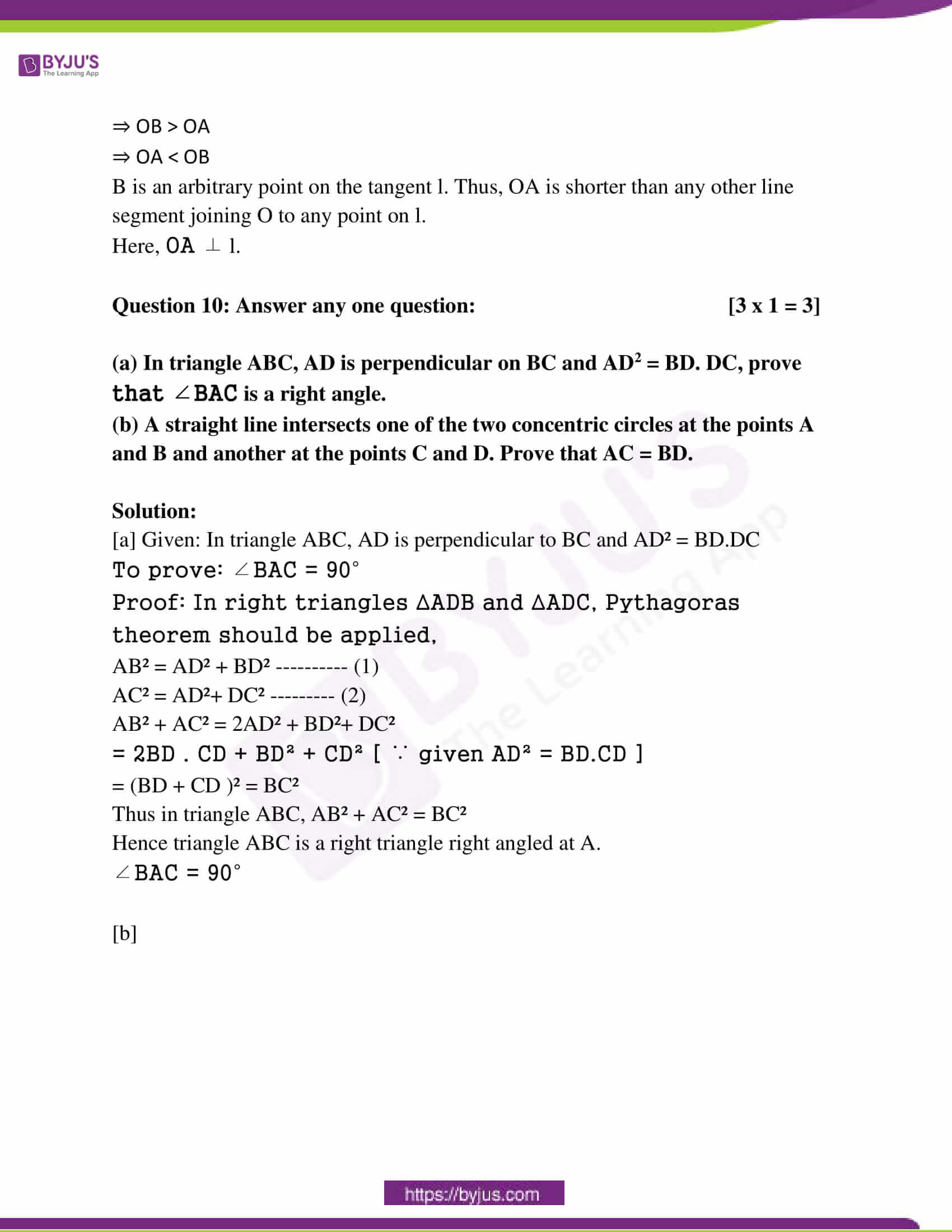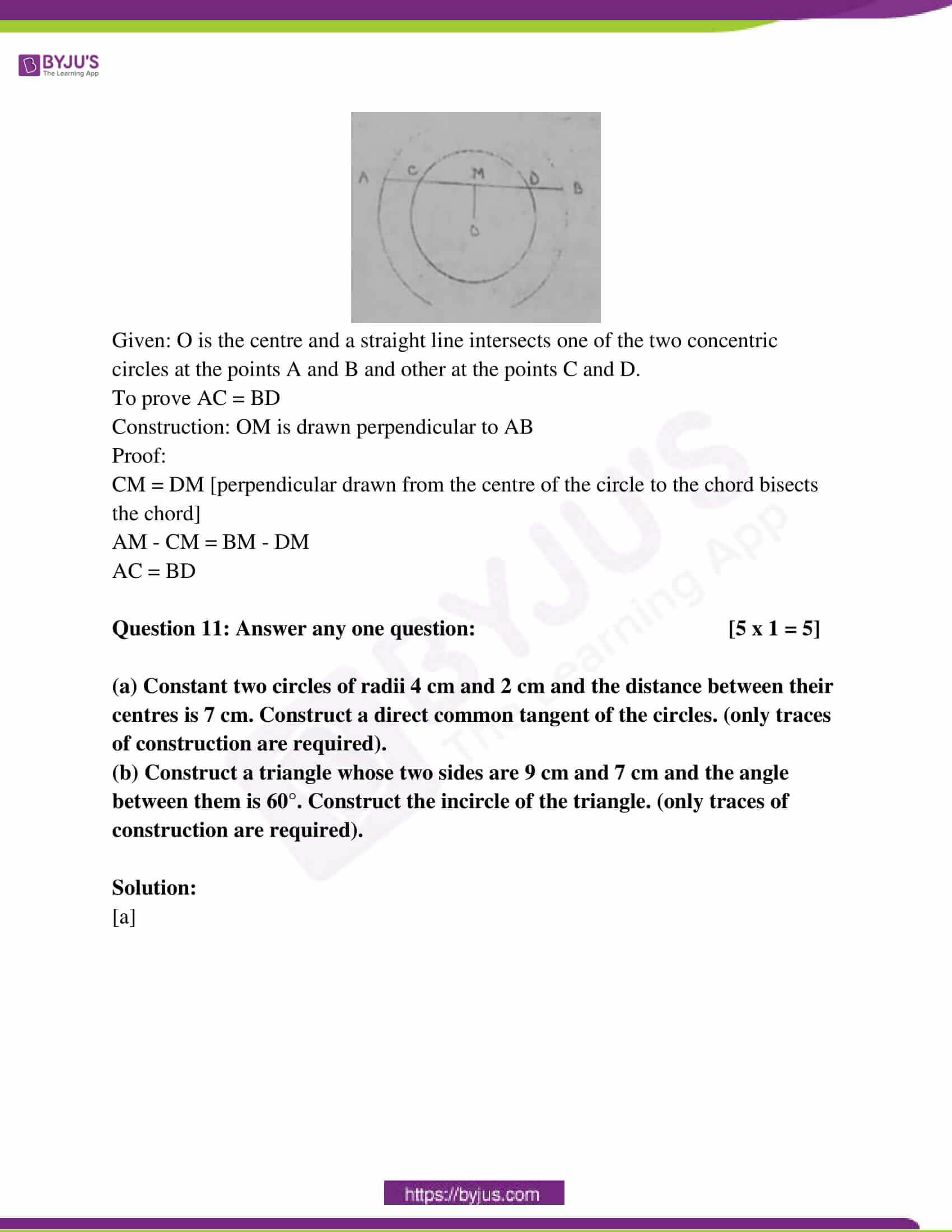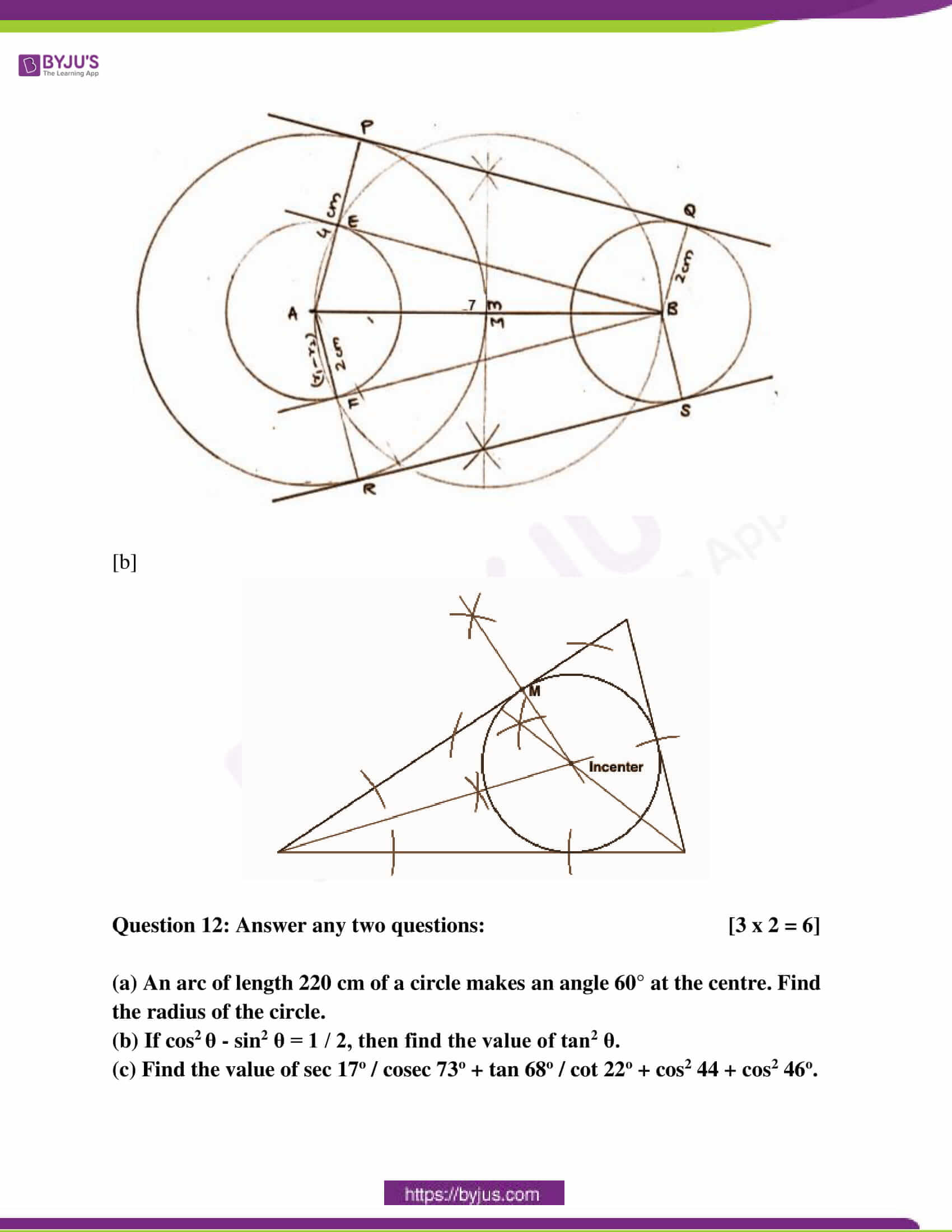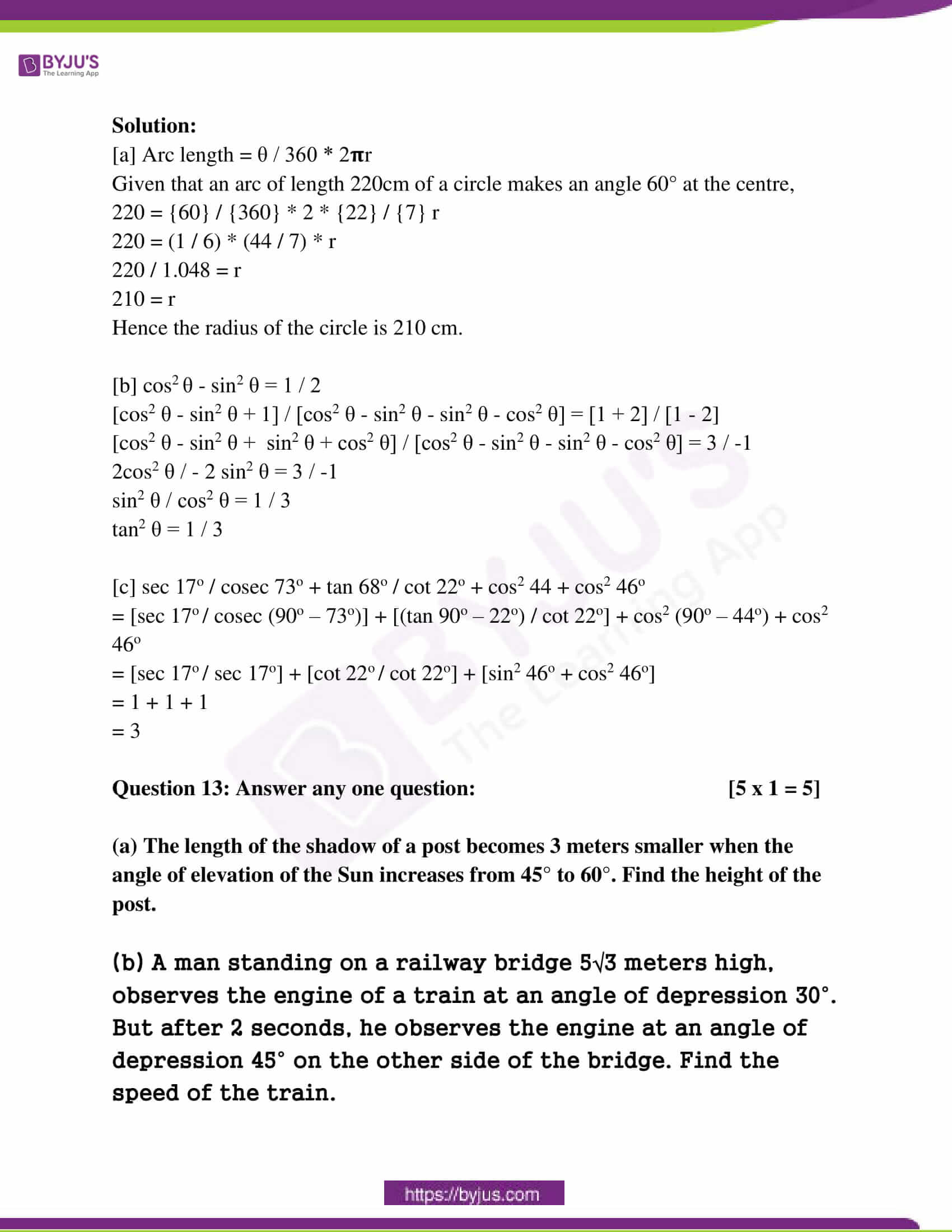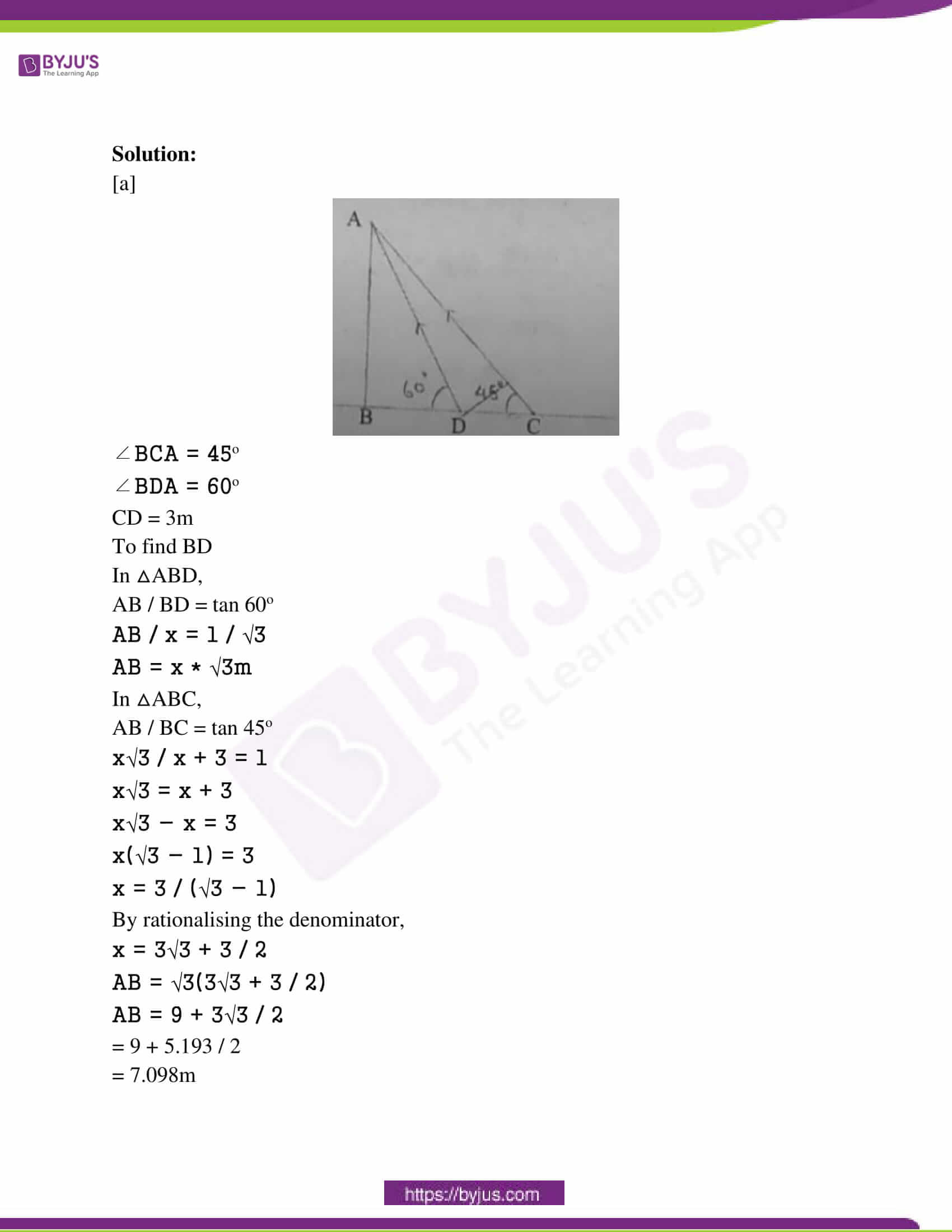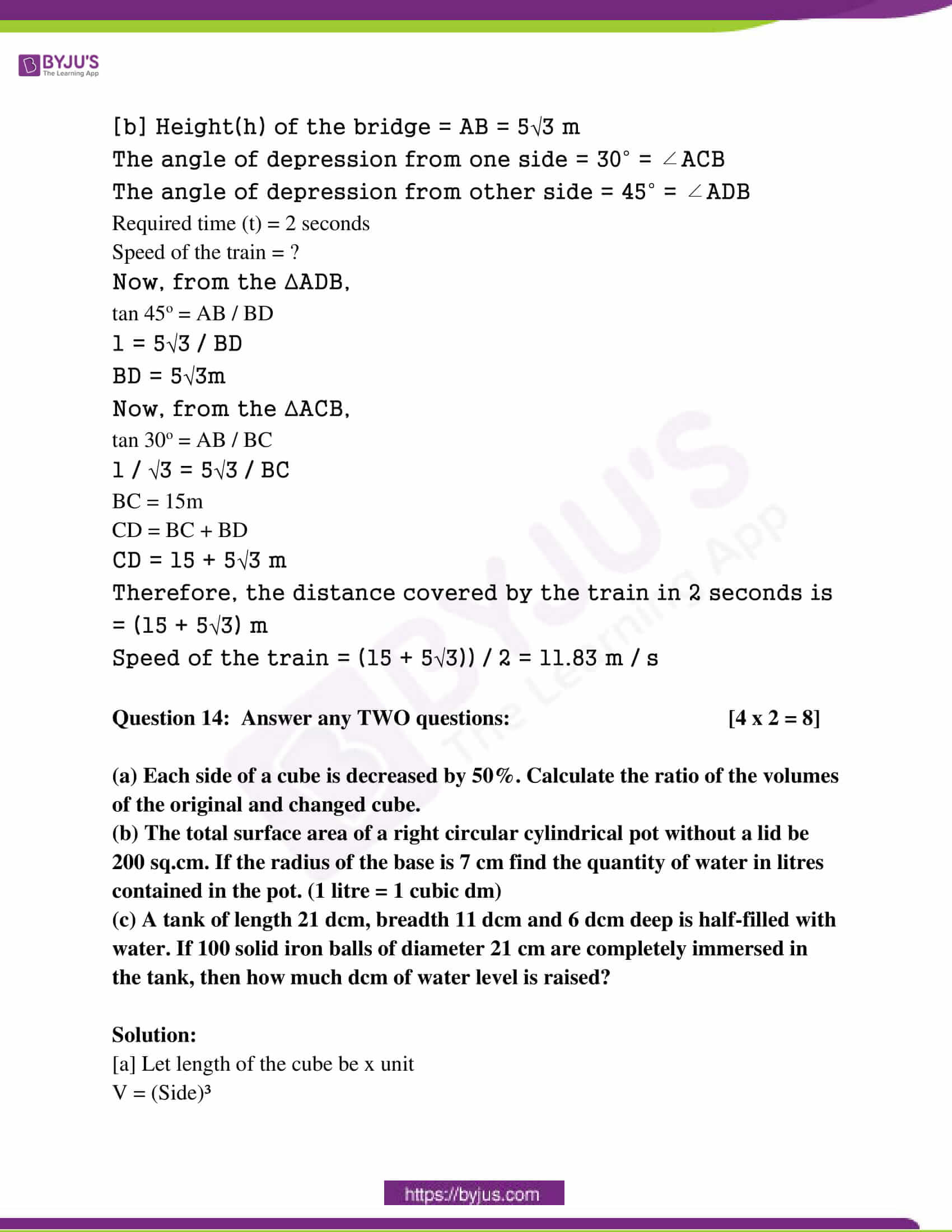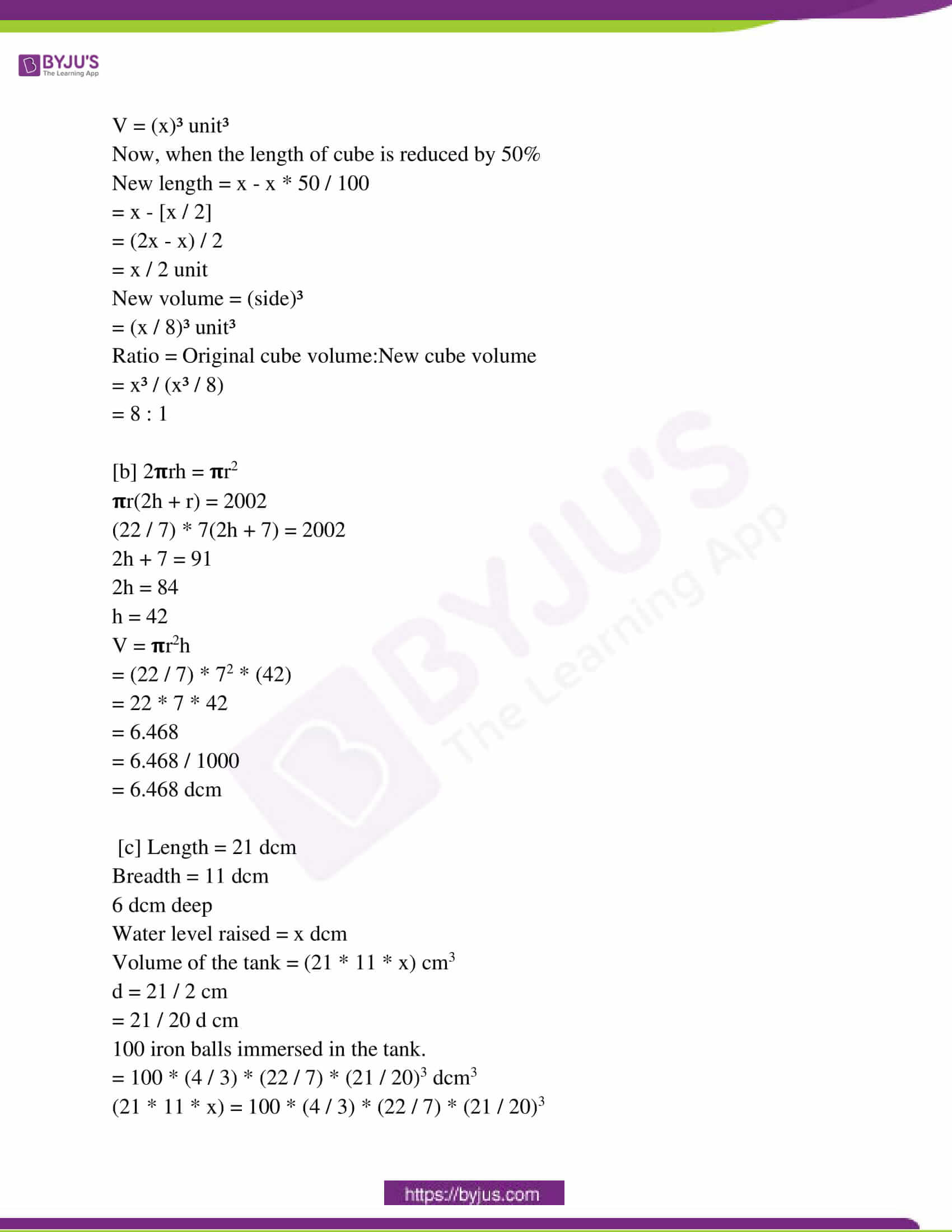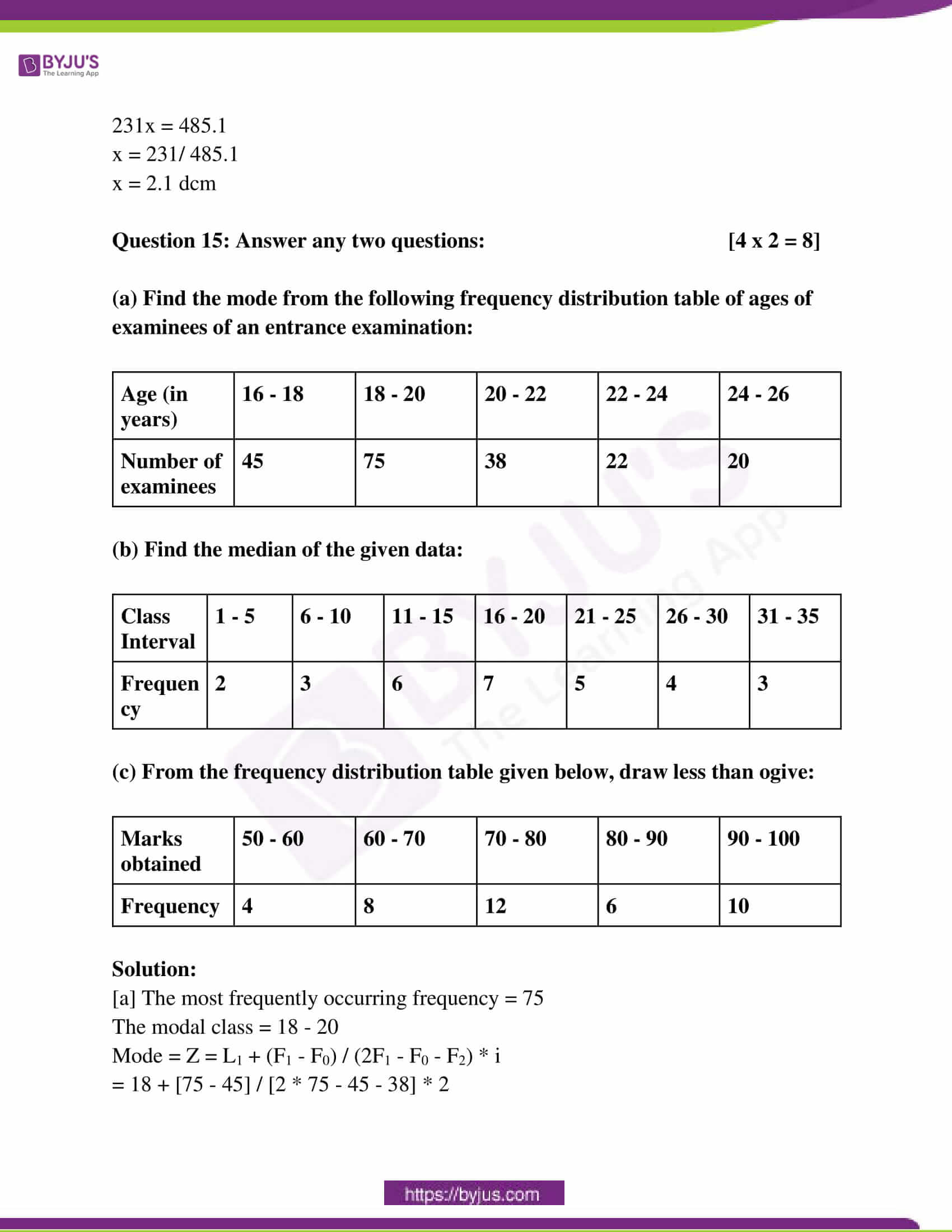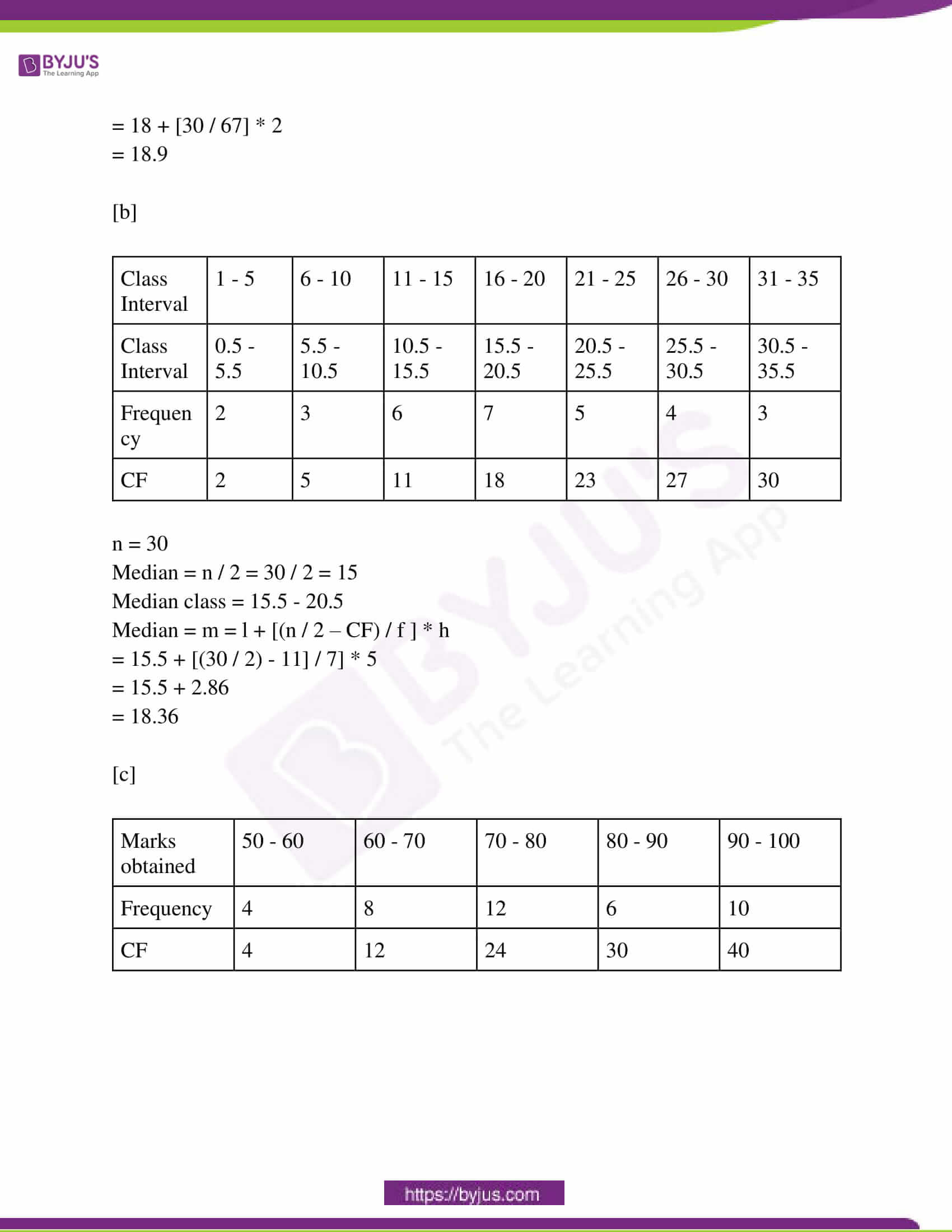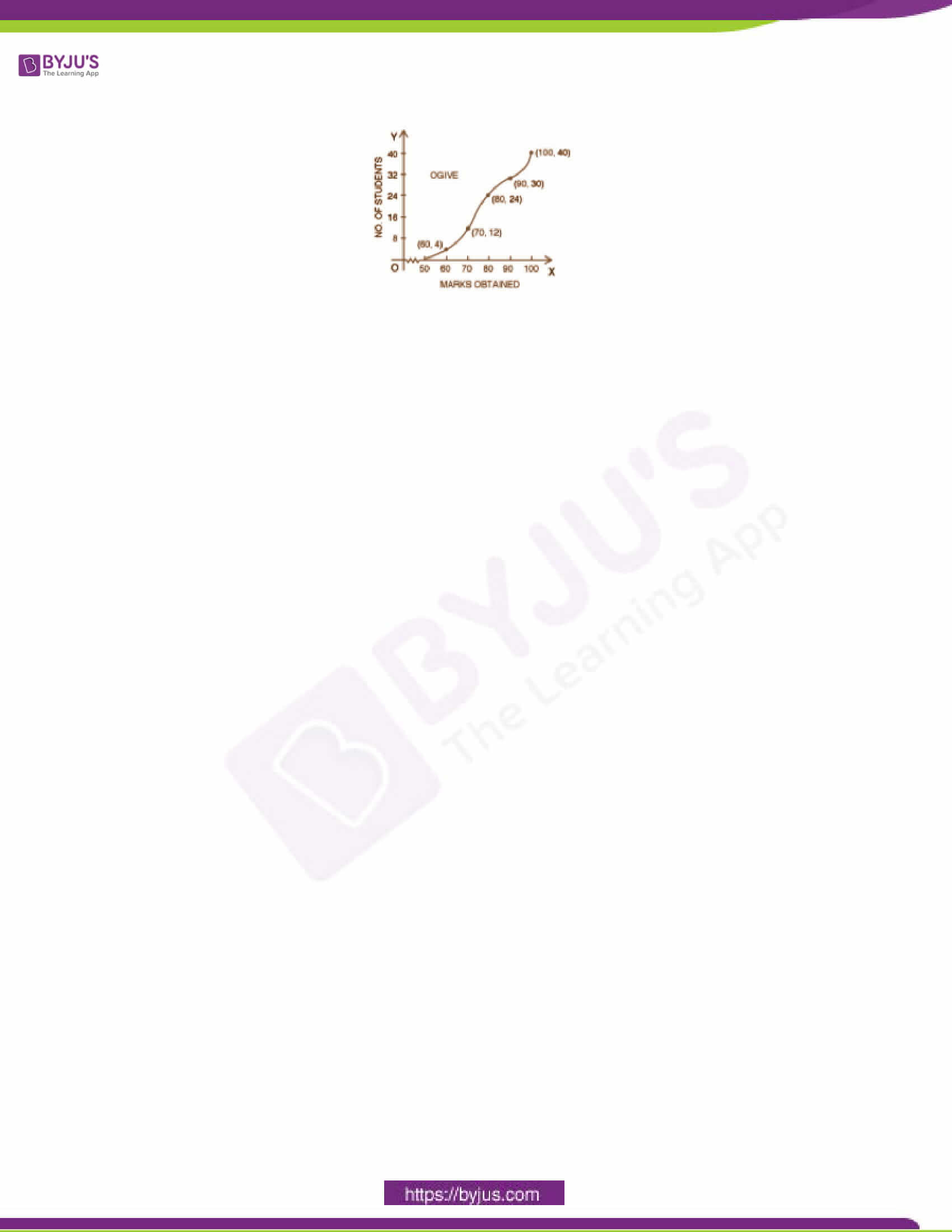Question 1: Choose the correct option in each case from the following questions: [1 x 6 = 6]

(i) Interest on Rs. a at the simple interest 10% per annum for b months is:

(a) Rs. ab / 100 (b) Rs. ab / 120 (c) Rs. ab / 1200 (d) Rs. ab / 10

R = 10%

T = b months = b / 12 years

SI = PTR / 100

= a * b * (10) / 100 * 12

= ab / 120

(ii) If x ∝ y then

(a) x2 ∝ y2 (b) x3 ∝ y2 (c) x ∝ y2 (d) x2 ∝ y2

x ∝ y

x = ky

x2 = k2y2

x2 ∝ y2

(iii) If ∠A = 100° of a cyclic quadrilateral ABCD, then the value of ∠C is:

(a) 50° (b) 20° (c) 80° (d) 180°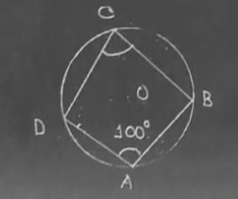∠A = 100°

∠A + ∠C = 180o

∠C = 180o – 100

∠C = 80o

(iv) The sexagesimal value of 7𝛑 / 12 is:

(a) 115° (b) 150° (c) 135° (d) 105°

7𝛑 / 12

= (7 * 180) / 12

= 105o

(v) If the side of a cube is a unit and the diagonal of the cube is d unit then the relation between a and d will be.

(a) √2a = d (b) √3a = d (c) a = √3d (d) a = √2d

(vi) If the mean of the numbers 6, 7, x, 8, y, 16 is 9 then:

(a) x + y = 21 (b) x + y = 17 (c) x – y = 21 (d) x – y = 9

6, 7, x, 8, y, 16 is 9

Mean = 9

9 = [6 + 7 + x + 8 + y + 16] / 6

54 = 37 + x + y

x + y = 17

Question 2: Fill up the blanks (any five): [1 x 5 = 5]

(i) If the simple interest of a principal for n years at r% p.a. be Rs. pnr / 25, then the principal will be Rs ______. [4P]

(ii) The equation (a – 2) x2 + 3x + 5 = 0 will not be a quadratic equation for a = ______. [a = 2]

(iii) if ABCD is a cyclic parallelogram then A is ______. [90o]

(iv) If tan 35° tan 55°= sin θ, then the lowest positive value of θ will be _______. [90o]

tan 35° tan 55°= sin θ

tan 35° tan (90o – 35o) = sin θ

tan 35o * cot 35o = sin θ

1 = sin θ

θ = 90o

(v) The shape of a pencil with one end sharpened is the combination of a cylinder and a _______ [cone]

(vi) The measures of central tendency are Mean, Median and ______. [Mode]

Question 3: Write True or False (any five): [1 x 5 = 5]

(i) At the same rate of interest, the simple interest for 2 years is more than the compound interest on the same principal. [False]

(ii) x3y, x2y2 and xy3 are in continued proportion. [True]

(iii) The angle in the segment of a circle which is less than a semicircle is an obtuse angle. [True]

(iv) Simplest value of sec227° – cot263° is 1. [True]

sec227° – cot263°

= sec227° – cot2 [90 – 27]

= sec227° – tan227°

= 1

(v) If the radius of a sphere is twice that of the 1st sphere then the volume of the sphere will be twice that of the 1st sphere. [False]

(vi)

 Score 1 2 3 4 5 Number of students 3 6 4 7 5

The mode of the distribution is 3. [False]

Question 4: Answer any one question: [3 x 1 = 3]

[i] The rate of simple interest per annum reduces from 4% to 3 (3 /4) % and for this, a person’s annual income decreases by Rs. 60. Determine the principal of that person.

Solution:

SI (1) = PTR / 100

= P * 4 * 1 / 100

= P / 25

Total income = P + (P / 25) = 26P / 25

SI (2) = PTR / 100

= P * (15 / 4) * 1 / 100

= 3P / 80

Total income = P + (3P / 80) = 83P / 80

[26P / 25] – 60 = 83P / 80

26P – 1500 / 25 = 83P / 80

415P = 416P – 24000

P = 24000

[ii] A and B start a business with Rs. 15,000 and Rs. 45,000, respectively. After 6 months B received Rs 3,030 as profit. What is A’s profit?

Solution:

Amount invested by A = Rs. 15000

Amount invested by B = Rs. 45000

The ratio of their profits after 6 months would be

A:B

15000:45000

15:45

1:3

Profit earned by B = Rs. 3030

According to the question, it becomes,

3x = 3010

x = 3010 / 3

x = 1010

So, A’s profit after 6 months would be Rs. 1010.

[iii] If 2x + [1 / x] = 2, then find the value of x / [2x2 + x + 1].

Solution:

2x + [1 / x] = 2

2x2 + 1 = 2x

LHS = x / [2x2 + x + 1]

= x / 2x2 + 1 + x

= x / 2x + x

= x / 3x

= 1 / 3

[iv] If the roots of a quadratic equation are 2 and -3, then write the equation.

Solution:

a = 2, b = -3

x2 – (a + b) x + ab = 0

x2 – (2 + (-3))x + (2 * -3) = 0

x2 + x – 6 = 0

[v] The line parallel to BC of △ABC meets AB and AC at P and Q respectively. If AP = 4 cm, QC = 9 cm and PB = AQ, then find the length of PB.

Solution: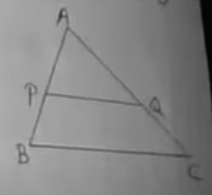Since PQ || BC

By basic proportionality theorem,

AP / PB = AQ / QC

4 / x = x / 9

36 = x2

x = 6cm

Length of PB is 6cm.

[vi] The radius of a circle with centre O is 5 cm. P is a point at a distance 13 cm from O. PQ and PR are two tangents to this circle. Find the area of the quadrilateral PQOR.

Solution: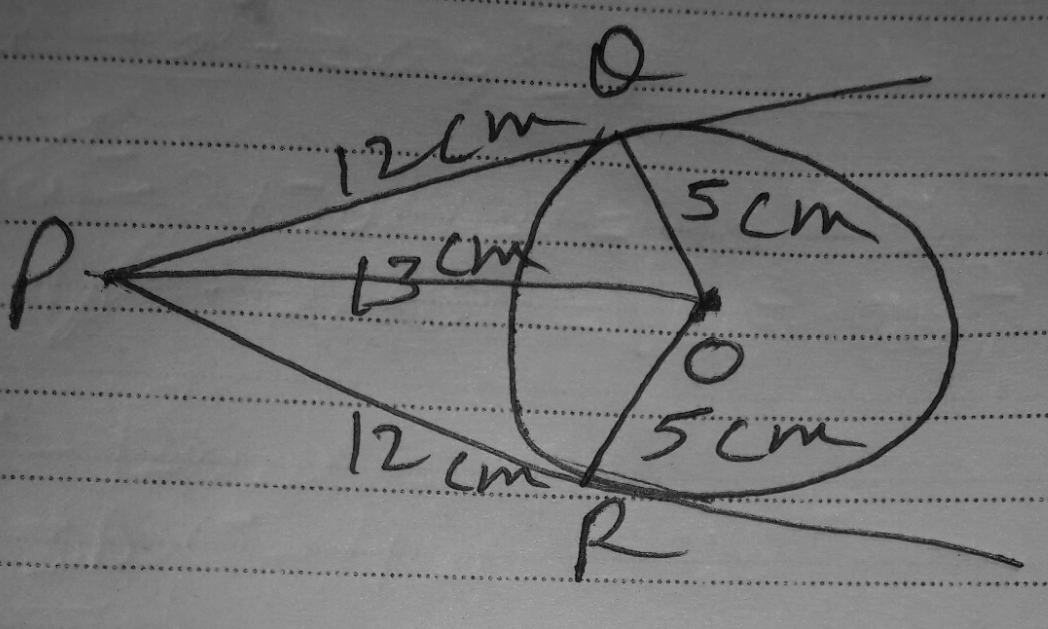PQ & PR are 2 tangents and QO & OR are 2 radii at contact point Q & R.

PQO = 90° [a tangent to a circle is perpendicular to the radius through the point of contact]

By Pythagoras theorem

PQ² = OP² – OQ²

PQ² = 13² – 5²

= 169 – 25

= 144

PQ = √144 = 12

PQ = 12cm

PQ = PR = 12cm [The lengths of two tangents drawn from an external point to a circle are equal]

In ∆OPQ & ∆ OPR

OQ = OR (5cm) given

OP = OP (Common)

PQ = PR (12cm)

Hence, ∆OPQ and ∆OPR are congruent. ( by SSS congruence)

Area of ∆OPQ = Area ∆OPR

Area of quadrilateral QORP = 2 × (area of ∆ OPR)

Area of quadrilateral QORP = 2 × 1 / 2 × base × altitude

Area of quadrilateral QORP = OR × PR

Area of quadrilateral QORP =12 × 5

= 60 cm²

[vii] The two chords AB and CD of a circle are at equal distance from the centre O. If ∠AOB = 60° and CD = 6 cm, then calculate the length of the radius of the circle.

Solution: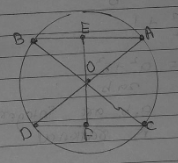In △AOB and △COD,

AB = CD

OA = OC = OB = OD

All the angles and sides should be equal.

AB = 6cm

∠COD = 60o

AE = AB / 2

AE = 6 / 2 = 3cm

In △AOE and △BOE,

OA = OB

OE = OE

AE = BE

By SSS congruence,

△AOE ⩭ △BOE

∠AOE = ∠BOE

∠AOE = 30o

sin θ = perpendicular / hypotenuse

sin 30o = AE / OA

[1 / 2] = AE / OA

OA = 3 * 2

OA = 6cm

[viii] If tan θ + cot θ = 2, then find the value of tan7θ + cot7θ.

Solution:

tan θ + cot θ = 2

⇒ tan θ + 1 / tan θ = 2

⇒ tan² θ + 1 = 2tan θ

⇒ tan² θ – 2tan θ + 1 = 0

⇒ (tan θ – 1)² = 0

⇒ tan θ = 1

cot θ = 1 / tan θ = 1

tan7 θ + cot7 θ

= (tan θ)7 + (cot θ)7

= (1)7 + (1)7

= 1 + 1

= 2

[ix] If the ratio of the length of the shadow of a tower and height of the tower is √3:1, find the angle of elevation of the sun.

Solution:

The ratio of the height of a tower and the length of its shadow is given by √3:1.

tan θ = Height of tower / Length of shadow

tanθ = √3 / 1

tan θ = tan 60o

θ = 60o

Hence, the angle of the elevation of the sun is 60°.

[x] The volumes of two right circular cylinders are the same. The ratio of their height is 1:2. Find the ratio of their radii.

Solution:

The volume of a right circular cylinder with radius r and height h is V = πr2h.

It is given that the ratio of the heights of two circular cylinders is 1:2 that is h1 / h2

= 1 / 2

V1 = V2

⇒ πr12h1 = πr22h2

⇒ r12 / r22 = h2 / h1

⇒ r12 / r22 = 1 / [h1 / h2]

⇒ r12 / r22 = 1 / (1 / 2)

⇒ (r1 / r2)2 = 2

⇒ r1 / r2 = √2

Hence, the ratio of their radius is √2:1.

[xi] The volume of a solid hemisphere is 144 π cubic cm, then find the diameter of the sphere.

Solution:

The volume of the hemisphere = 2πr3 / 3

144π = 2 * (22 / 7) * r3 / 3

144 * 3 = 2 * r3

216 = r3

r = 6cm

d = 2 * r = 2 * 6 = 12cm

[xii] The mean of a frequency distribution is 8.1 if ∑fixi = 132 + 5K and ∑fi = 20 then what is the value of K?

Solution:

Mean = ∑fixi / ∑fi

Mean = 8.1

∑fixi = 132 + 5K

∑fi = 20

8.1 = (132 + 5k) / 20

8.1 × 20 = 132 + 5k

162 = 132 + 5k

162 – 132 = 5k

30 = 5k

k = 6

Question 5: Answer any one question : [5 x 1 = 5]

(a) Aminur has taken a loan of Rs. 64, 000 from a bank. If the rate of interest is 2.5 paise per rupee per annum, calculate the compound interest payable after 2 years.

(b) A, B and C start a business with the capital of Rs. 6,000, Rs 8,000 and Rs. 9, 000, respectively. After a few months, A invests Rs 3, 000 more in the business. At the end of the year, they gained Rs 30,000 and C got Rs. 10,800 as a share of profit. When did A invest Rs. 3,000 more?

Solution:

[a] P = Rs. 64000

r = 2.5 paise per rupee per annum (given)

= 0.025 rupee per rupee per annum

= 0.025 x 100 rupee per hundred rupee per annum

= 0.025 x 100 per cent per annum

= 2.5 percent per annum

t = 2 years

C.I. = 64000 [(1 + 2.5 / 100)2]

= 64000 [(1.025)2]

= 64000 x 1.050625

= 67240

= Rs. 67240

CI = 67240 – 64000 = Rs. 3240

[b] A invests Rs 3, 000 more in the business.

A = 6000 + 3000 = 9000

= 6000 * x + 9000 (12 – x)

= 6000x + 108000 – 9000x

= 108000 – 3000x

= 3000 (36 – x)

B invested Rs. 8000

= (8000 * 12)

= Rs. 96000

C invested Rs. 9000

= (9000 * 12)

= Rs. 108000

Ratio of A, B and C together

= 3000 (36 – x) : 96000 : 108000

= (36 – x) : 32 : 36

Gain = Rs. 30000

C = 30000 * [36 / (36 – x) + 32 + 36]

= 30000 * [36 / 104 – x]

30000 * [36 / 104 – x] = 10800

36 / 104 – x = 10800 / 30000

936 – 9x = 900

-9x = -36

x = 4

Question 6: Answer any one question: [3 x 1 = 3]

(a) Solve: {[x + 4] / [x – 4]}2 – 5 [x + 4 / x – 4] + 6 = 0, (x ≠ 4)

(b) The digit in the unit’s place of a two-digit number is 6 more than that at the ten’s place. The product of the digits is 12 less than the number. Find the possible values of the digit in the unit place.

Solution:

[a] {[x + 4] / [x – 4]}2 – 5 [x + 4 / x – 4] + 6 = 0,

Take [x + 4 / x – 4] = a

a2 – 5a + 6 = 0

a2 – 3a – 2a + 6 = 0

a (a – 3) – 2 (a – 3) = 0

(a – 3) (a – 2) = 0

a = 3, 2

a = 3

x + 4 / x – 4 = 3

x + 4 = 3 (x – 4)

x + 4 = 3x – 12

12 + 4 = 3x – x

16 = 2x

x = 8

a = 2

x + 4 / x – 4 = 2

x + 4 = 2 (x – 4)

x + 4 = 2x – 8

8 + 4 = 2x – x

12 = x

[b] x (x + 6) = 10x + x + 6 – 12

x2 + 6x = 11x – 6

x2 – 5x + 6 = 0

(x – 3) (x – 2) = 0

x = 3, 2

3 + 6 = 9

2 + 6 = 8

Question 7: Answer any one question: [3 x 1 = 3]

(a) Find the simplest value of √7 (√5 – √2) – √5 (√7 – √2) + 2√2 / √5 + √7.

(b) If x ∝ y and y ∝ z, then prove that: (x2 + y2 + z2) ∝ (xy + yz + xz)

Solution:

[a] √7 (√5 – √2) – √5 (√7 – √2) + 2√2 / √5 + √7

= √35 – √14 – √35 + √10 + [2√2 (√7 – √5) / (√5 + √7) (√7 – √5)]

= √35 – √14 – √35 + √10 + 2√2 (√7 – √5) / (√7)2 – (√5)2

= √35 – √14 – √35 + √10 + 2√2 (√7 – √5) / 2

= √35 – √14 – √35 + √10 + √14 – √10

= 0

[b] x ∝ y

x = k1y

y ∝ z

y = k2z

Hence, x = k1k2z

(x2 + y2 + z2) ∝ (xy + yz + xz)

= (k1k2z)2 + (k2z)2 + z2 / [k1y * k2z + k2z * z + k1y * z]

= z2 (k12 k22 + k22 + 1) / [k1k22z2 + k2z2 + k1k2z2]

= z2 (k12 k22 + k22 + 1) / z2 [k1k22 + k2 + k1k2]

= (k12 k22 + k22 + 1) / [k1k22 + k2 + k1k2]

So, (x2 + y2 + z2) ∝ (xy + yz + xz)

Question 8: Answer any one question: [3 x 1 = 3]

[a] If [a + b – c] / [a + b] = [b + c – a] / [b + c] = [c + a – b] / [c + a] and a + b + c ≠ 0 then prove that a + b = c.

[b] If x:a, y:b, z:c that show that (a2 + b2 + c2) (x2 + y2 + z2) = (ax + by + cz)2.

Solution:

[a] [a + b – c] / [a + b] = [b + c – a] / [b + c] = [c + a – b] / [c + a] [a + b] / [a + b] – [c] / [a + b] = [b + c] / [b + c] – [a] / [b + c] = [c + a] / [c + a] – [b] / [c + a]

1 – [c] / [a + b] = 1 – [a] / [b + c] = 1 – [b] / [c + a] [c] / [a + b] = [a] / [b + c] = [b] / [c + a]

a + b / c = b + c / a = c + a / b

a + b + c / c = b + c + a / a = c + a + b / b

1 / c = 1 / a = 1 / b

c = a = b or a = b = c

[b] x:a, y:b, z:c

x / a = y / b = z / c = k [Say]

Let x = ka, y = kb, z = kc

LHS = (a2 + b2 + c2) (x2 + y2 + z2)

= (a2 + b2 + c2) (k2a2 + k2b2 + k2c2)

= k2 (a2 + b2 + c2) (a2 + b2 + c2)

= k2 (a2 + b2 + c2)2

RHS = (ax + by + cz)2

= (a * ka + b * kb + c * kc)2

= (k2a2 + k2b2 + k2c2)2

= k2 (a2 + b2 + c2)2

Question 9: Answer any one question: [5 x 1 = 5]

(a) Prove that, if a perpendicular is drawn on the hypotenuse from the right angular point of a right-angled triangle, two triangles so formed on the two sides of the perpendicular are each similar to the original triangle and also similar to each other.

(b) Prove that the tangent and the radius through the point of contact of a circle are perpendicular to each other.

Solution:

[a]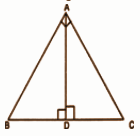Given a right angle triangle, right-angled at A.

AD is the perpendicular drawn to the hypotenuse BC from vertex A.

To Prove:

(i) ∆BDA ~ ∆BAC

Proof:

In ∆BDA and ∆BAC:

∠B = ∠B [common]

Therefore, by using AA similar condition,

∆BDA ~ ∆BAC …(i)

∠C = ∠C [common]

Therefore, by using AA similar condition,

Comparing (i) and (ii), ∆BDA ~ ∆ADC.

[b]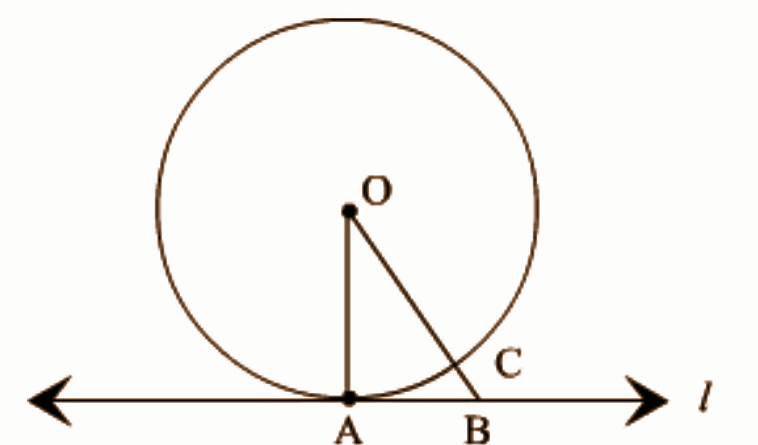Given: A circle C (0, r) and a tangent l at point A.

To prove: OA ⊥ l

Construction: Take a point B, other than A, on the tangent l. Join OB. Suppose OB meets the circle in C.

Proof: We know that, among all line segments joining the point O to a point on l, the perpendicular is shortest to l.

OA = OC (Radius of the same circle)

Now, OB = OC + BC.

∴ OB > OC

⇒ OB > OA

⇒ OA < OB

B is an arbitrary point on the tangent l. Thus, OA is shorter than any other line segment joining O to any point on l.

Here, OA ⊥ l.

Question 10: Answer any one question: [3 x 1 = 3]

(a) In triangle ABC, AD is perpendicular on BC and AD2 = BD. DC, prove that ∠BAC is a right angle.

(b) A straight line intersects one of the two concentric circles at the points A and B and another at the points C and D. Prove that AC = BD.

Solution:

[a] Given: In triangle ABC, AD is perpendicular to BC and AD² = BD.DC

To prove: ∠BAC = 90°

Proof: In right triangles ∆ADB and ∆ADC, Pythagoras theorem should be applied,

AB² = AD² + BD² ———- (1)

AC² = AD²+ DC² ——— (2)

AB² + AC² = 2AD² + BD²+ DC²

= 2BD . CD + BD² + CD² [ ∵ given AD² = BD.CD ]

= (BD + CD )² = BC²

Thus in triangle ABC, AB² + AC² = BC²

Hence triangle ABC is a right triangle right angled at A.

∠BAC = 90°

[b]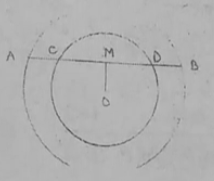Given: O is the centre and a straight line intersects one of the two concentric circles at the points A and B and other at the points C and D.

To prove AC = BD

Construction: OM is drawn perpendicular to AB

Proof:

CM = DM [perpendicular drawn from the centre of the circle to the chord bisects the chord]

AM – CM = BM – DM

AC = BD

Question 11: Answer any one question: [5 x 1 = 5]

(a) Constant two circles of radii 4 cm and 2 cm and the distance between their centres is 7 cm. Construct a direct common tangent of the circles. (only traces of construction are required).

(b) Construct a triangle whose two sides are 9 cm and 7 cm and the angle between them is 60°. Construct the incircle of the triangle. (only traces of construction are required).

Solution:

[a]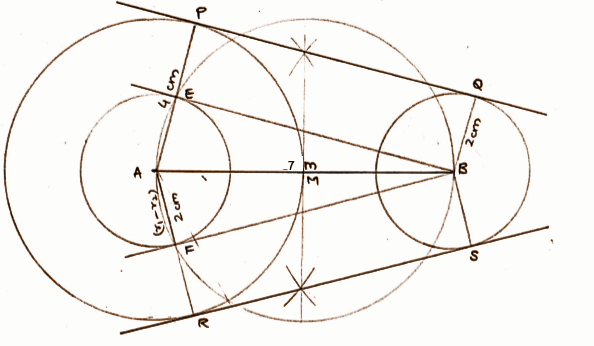[b]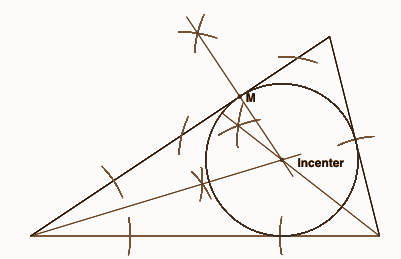Question 12: Answer any two questions: [3 x 2 = 6]

(a) An arc of length 220 cm of a circle makes an angle 60° at the centre. Find the radius of the circle.

(b) If cos2 θ – sin2 θ = 1 / 2, then find the value of tan2 θ.

(c) Find the value of sec 17o / cosec 73o + tan 68o / cot 22o + cos2 44 + cos2 46o.

Solution:

[a] Arc length = θ / 360 * 2𝛑r

Given that an arc of length 220cm of a circle makes an angle 60° at the centre,

220 = {60} / {360} * 2 * {22} / {7} r

220 = (1 / 6) * (44 / 7) * r

220 / 1.048 = r

210 = r

Hence the radius of the circle is 210 cm.

[b] cos2 θ – sin2 θ = 1 / 2

[cos2 θ – sin2 θ + 1] / [cos2 θ – sin2 θ – sin2 θ – cos2 θ] = [1 + 2] / [1 – 2] [cos2 θ – sin2 θ + sin2 θ + cos2 θ] / [cos2 θ – sin2 θ – sin2 θ – cos2 θ] = 3 / -1

2cos2 θ / – 2 sin2 θ = 3 / -1

sin2 θ / cos2 θ = 1 / 3

tan2 θ = 1 / 3

[c] sec 17o / cosec 73o + tan 68o / cot 22o + cos2 44 + cos2 46o

= [sec 17o / cosec (90o – 73o)] + [(tan 90o – 22o) / cot 22o] + cos2 (90o – 44o) + cos2 46o

= [sec 17o / sec 17o] + [cot 22o / cot 22o] + [sin2 46o + cos2 46o]

= 1 + 1 + 1

= 3

Question 13: Answer any one question: [5 x 1 = 5]

(a) The length of the shadow of a post becomes 3 meters smaller when the angle of elevation of the Sun increases from 45° to 60°. Find the height of the post.

(b) A man standing on a railway bridge 5√3 meters high, observes the engine of a train at an angle of depression 30°. But after 2 seconds, he observes the engine at an angle of depression 45° on the other side of the bridge. Find the speed of the train.

Solution:

[a]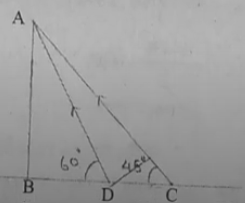∠BCA = 45o

∠BDA = 60o

CD = 3m

To find BD

In △ABD,

AB / BD = tan 60o

AB / x = 1 / √3

AB = x * √3m

In △ABC,

AB / BC = tan 45o

x√3 / x + 3 = 1

x√3 = x + 3

x√3 – x = 3

x(√3 – 1) = 3

x = 3 / (√3 – 1)

By rationalising the denominator,

x = 3√3 + 3 / 2

AB = √3(3√3 + 3 / 2)

AB = 9 + 3√3 / 2

= 9 + 5.193 / 2

= 7.098m

[b] Height(h) of the bridge = AB = 5√3 m

The angle of depression from one side = 30° = ∠ACB

The angle of depression from other side = 45° = ∠ADB

Required time (t) = 2 seconds

Speed of the train = ?

tan 45o = AB / BD

1 = 5√3 / BD

BD = 5√3m

Now, from the ∆ACB,

tan 30o = AB / BC

1 / √3 = 5√3 / BC

BC = 15m

CD = BC + BD

CD = 15 + 5√3 m

Therefore, the distance covered by the train in 2 seconds is = (15 + 5√3) m

Speed of the train = (15 + 5√3)) / 2 = 11.83 m / s

Question 14: Answer any TWO questions: [4 x 2 = 8]

(a) Each side of a cube is decreased by 50%. Calculate the ratio of the volumes of the original and changed cube.

(b) The total surface area of a right circular cylindrical pot without a lid be 200 sq.cm. If the radius of the base is 7 cm find the quantity of water in litres contained in the pot. (1 litre = 1 cubic dm)

(c) A tank of length 21 dcm, breadth 11 dcm and 6 dcm deep is half-filled with water. If 100 solid iron balls of diameter 21 cm are completely immersed in the tank, then how much dcm of water level is raised?

Solution:

[a] Let length of the cube be x unit

V = (Side)³

V = (x)³ unit³

Now, when the length of cube is reduced by 50%

New length = x – x * 50 / 100

= x – [x / 2]

= (2x – x) / 2

= x / 2 unit

New volume = (side)³

= (x / 8)³ unit³

Ratio = Original cube volume:New cube volume

= x³ / (x³ / 8)

= 8 : 1

[b] 2𝛑rh = 𝛑r2

𝛑r(2h + r) = 2002

(22 / 7) * 7(2h + 7) = 2002

2h + 7 = 91

2h = 84

h = 42

V = 𝛑r2h

= (22 / 7) * 72 * (42)

= 22 * 7 * 42

= 6.468

= 6.468 / 1000

= 6.468 dcm

[c] Length = 21 dcm

6 dcm deep

Water level raised = x dcm

Volume of the tank = (21 * 11 * x) cm3

d = 21 / 2 cm

= 21 / 20 d cm

100 iron balls immersed in the tank.

= 100 * (4 / 3) * (22 / 7) * (21 / 20)3 dcm3

(21 * 11 * x) = 100 * (4 / 3) * (22 / 7) * (21 / 20)3

231x = 485.1

x = 231/ 485.1

x = 2.1 dcm

Question 15: Answer any two questions: [4 x 2 = 8]

(a) Find the mode from the following frequency distribution table of ages of examinees of an entrance examination:

 Age (in years) 16 – 18 18 – 20 20 – 22 22 – 24 24 – 26 Number of examinees 45 75 38 22 20

(b) Find the median of the given data:

 Class Interval 1 – 5 6 – 10 11 – 15 16 – 20 21 – 25 26 – 30 31 – 35 Frequency 2 3 6 7 5 4 3

(c) From the frequency distribution table given below, draw less than ogive:

 Marks obtained 50 – 60 60 – 70 70 – 80 80 – 90 90 – 100 Frequency 4 8 12 6 10

Solution:

[a] The most frequently occurring frequency = 75

The modal class = 18 – 20

Mode = Z = L1 + (F1 – F0) / (2F1 – F0 – F2) * i

= 18 + [75 – 45] / [2 * 75 – 45 – 38] * 2

= 18 + [30 / 67] * 2

= 18.9

[b]
 Class Interval 1 – 5 6 – 10 11 – 15 16 – 20 21 – 25 26 – 30 31 – 35 Class Interval 0.5 – 5.5 5.5 – 10.5 10.5 – 15.5 15.5 – 20.5 20.5 – 25.5 25.5 – 30.5 30.5 – 35.5 Frequency 2 3 6 7 5 4 3 CF 2 5 11 18 23 27 30

n = 30

Median = n / 2 = 30 / 2 = 15

Median class = 15.5 – 20.5

Median = m = l + [(n / 2 – CF) / f ] * h

= 15.5 + [(30 / 2) – 11] / 7] * 5

= 15.5 + 2.86

= 18.36

[c]
 Marks obtained 50 – 60 60 – 70 70 – 80 80 – 90 90 – 100 Frequency 4 8 12 6 10 CF 4 12 24 30 40